SNAS411P August   2008  – April 2017

PRODUCTION DATA.

1. Features
2. Applications
3. Description
4. Revision History
5. Pin Configuration and Functions
6. Specifications
7. Detailed Description
1. 7.1 Overview
2. 7.2 Functional Block Diagram
3. 7.3 Feature Description
4. 7.4 Device Functional Modes
5. 7.5 Programming
8. Application and Implementation
1. 8.1 Application Information
2. 8.2 Typical Application
9. Power Supply Recommendations
10. 10Layout
11. 11Device and Documentation Support
1. 11.1 Device Support
1. 11.1.1 Development Support
2. 11.1.2 Device Nomenclature
2. 11.2 Receiving Notification of Documentation Updates
3. 11.3 Community Resources
5. 11.5 Electrostatic Discharge Caution
6. 11.6 Glossary
12. 12Mechanical, Packaging, and Orderable Information

• NAC|16
• Y|0

## 6 Specifications

### 6.1 Absolute Maximum Ratings(1)

MINMAXUNIT
VAAnalog supply voltage–0.36.5V
VDDigital supply voltage(4)–0.3VA + 0.3V
Voltage on any pin to GND–0.3VA + 0.3V
Input current at any pin (2)±10mA
Power dissipation TA = 25°CSee (3)
Package input current(2)±20 mAmA
Soldering temperature, 10 seconds 260°C
Junction temperature175°C
TstgStorage temperature–65150°C
Stresses beyond those listed under Absolute Maximum Ratings may cause permanent damage to the device. These are stress ratings only, and functional operation of the device at these or any other conditions beyond those indicated under Recommended Operating Conditions is not implied. Exposure to absolute-maximum-rated conditions for extended periods may affect device reliability.
When the input voltage at any pin exceeds the power supplies (that is, VIN less than AGND or VIN greater than VA or VD), the current at that pin should be limited to 10 mA. The 20 mA maximum package input current rating limits the number of pins that can safely exceed the power supplies with an input current of 10 mA to two.
The absolute maximum junction temperature (TJmax) for this device is 175°C. The maximum allowable power dissipation is dictated by TJmax, the junction-to-ambient thermal resistance (RθJA), and the ambient temperature (TA), and can be calculated using the formula PDMAX = (TJmax − TA)/RθJA. The values for maximum power dissipation listed above will be reached only when the ADC128S102QML-SP is operated in a severe fault condition (for example, when input or output pins are driven beyond the power supply voltages, or the power supply polarity is reversed). Obviously, such conditions should always be avoided.
The maximum voltage is not to exceed 6.5 V

### 6.2 ESD Ratings

VALUEUNIT
V(ESD)Electrostatic dischargeHuman-body model (HBM), per ANSI/ESDA/JEDEC JS-001(1)(2) ±8000V
JEDEC document JEP155 states that 500-V HBM allows safe manufacturing with a standard ESD control process.
Human body model is 100-pF capacitor discharged through a 1.5-kΩ resistor. Machine model is 220 pF discharged through 0 Ω.

### 6.3 Recommended Operating Conditions

See (1)(2)
MINMAXUNIT
Operating temperature–55125°C
VA supply voltage2.75.25V
VD supply voltage2.7 VAV
Digital input voltage 0VAV
Clock frequency0.8 16MHz
Absolute Maximum Ratings indicate limits beyond which damage to the device may occur. Recommended Operating Conditions indicate conditions for which the device is functional, but do not verify specific performance limits. For specifications and test conditions, see the Electrical Characteristics. The specified specifications apply only for the test conditions listed. Some performance characteristics may degrade when the device is not operated under the listed test conditions.
All voltages are measured with respect to GND = 0 V, unless otherwise specified.

### 6.4 Thermal Information

THERMAL METRIC(1)ACD128S102QML-SPUNIT
NAC (CFP)
16 PINS
RθJAJunction-to-ambient thermal resistance 127°C/W
RθJC(top)Junction-to-case (top) thermal resistance 11.2°C/W
For more information about traditional and new thermal metrics, see the Semiconductor and IC Package Thermal Metrics application report.

### 6.5 Electrical Characteristics: ADC128S102QML-SP Converter

The following specifications apply for AGND = DGND = 0V, fSCLK = 0.8 MHz to 16 MHz, fSAMPLE = 50 kSPS to 1 MSPS, CL = 50pF, unless otherwise noted.
PARAMETERTEST CONDITIONSSUBGROUPMINTYP(1)MAXUNIT
STATIC CONVERTER CHARACTERISTICS
Resolution with no missing codes12Bits
INLIntegral non-linearity (end point method)VA = VD = 3 V[1, 2, 3]–1±0.61.1LSB
VA = VD = 5 V[1, 2, 3]–1.25±0.91.4LSB
DNLDifferential non-linearityVA = VD = 3 V[1, 2, 3]0.50.9LSB
[1, 2, 3]–0.7–0.3LSB
VA = VD = 5 V[1, 2, 3]0.91.5LSB
[1, 2, 3]–0.9−0.5LSB
VOFFOffset errorVA = VD = 3 V[1, 2, 3]–2.30.82.3LSB
VA = VD = 5 V[1, 2, 3]–2.31.12.3LSB
OEMOffset error matchVA = VD = 3 V[1, 2, 3]–1.5±0.11.5LSB
VA = VD = 5 V[1, 2, 3]–1.5±0.31.5LSB
FSEFull scale errorVA = VD = 3 V[1, 2, 3]–20.82LSB
VA = VD = 5 V[1, 2, 3]–20.32LSB
FSEMFull scale error matchVA = VD = 3 V[1, 2, 3]–1.5±0.11.5LSB
VA = VD = 5 V[1, 2, 3]–1.5±0.31.5LSB
DYNAMIC CONVERTER CHARACTERISTICS
FPBWFull power bandwidth (–3 dB)VA = VD = 3 V6.8MHz
VA = VD = 5 V10MHz
SINADSignal-to-noise plus distortion ratioVA = VD = 3 V,
fIN = 40.2 kHz, −0.02 dBFS
[4, 5, 6]6872dB
VA = VD = 5 V,
fIN = 40.2 kHz, −0.02 dBFS
[4, 5, 6]6872dB
SNRSignal-to-noise ratioVA = VD = 3 V,
fIN = 40.2 kHz, −0.02 dBFS
[4, 5, 6]6972dB
VA = VD = 5 V,
fIN = 40.2 kHz, −0.02 dBFS
[4, 5, 6]68.572dB
THDTotal harmonic distortionVA = VD = 3 V,
fIN = 40.2 kHz, −0.02 dBFS
[4, 5, 6]–86–74dB
VA = VD = 5 V,
fIN = 40.2 kHz, −0.02 dBFS
[4, 5, 6]–87–74dB
SFDRSpurious-free dynamic rangeVA = VD = 3 V,
fIN = 40.2 kHz, −0.02 dBFS
[4, 5, 6]7591dB
VA = VD = 5 V,
fIN = 40.2 kHz, −0.02 dBFS
[4, 5, 6]7590dB
ENOBEffective number of bitsVA = VD = 3 V,
fIN = 40.2 kHz
[4, 5, 6]11.111.6Bits
VA = VD = 5 V,
fIN = 40.2 kHz, −0.02 dBFS
[4, 5, 6]11.111.6Bits
ISOChannel-to-channel isolationVA = VD = 3 V,
fIN = 20 kHz
84dB
VA = VD = 5 V,
fIN = 20 kHz, −0.02 dBFS
85 dB
IMDIntermodulation distortion, second order termsVA = VD = 3 V,
fa = 19.5 kHz, fb = 20.5 kHz
[4, 5, 6]–93–78 dB
VA = VD = 5 V,
fa = 19.5 kHz, fb = 20.5 kHz
[4, 5, 6]–93–78 dB
Intermodulation distortion, third order termsVA = VD = 3 V,
fa = 19.5 kHz, fb = 20.5 kHz
[4, 5, 6]–91–70dB
VA = VD = 5 V,
fa = 19.5 kHz, fb = 20.5 kHz
[4, 5, 6]–91–70dB
VINInput range0 to VAV
IDCLDC leakage current[1, 2, 3]±0.01±1µA
CINAInput capacitanceTrack mode, see (2)38pF
Hold mode, see (2)4.5pF
DIGITAL INPUT CHARACTERISTICS
VIHInput high voltageVA = VD = 2.7 V to 3.6 V[1, 2, 3]2.1V
VA = VD = 4.75 V to 5.25 V[1, 2, 3]2.4V
VILInput low voltageVA = VD = 2.7 V to 5.25 V[1, 2, 3]0.8V
IINInput currentVIN = 0 V or VD[1, 2, 3]±1±1µA
CINDDigital input capacitanceSee (2)3.5pF
DIGITAL OUTPUT CHARACTERISTICS
VOHOutput high voltageISOURCE = 200 µA,
VA = VD = 2.7 V to 5.25 V
[1, 2, 3]VD –0.5V
VOLOutput low voltageISINK = 200 µA to 1 mA,
VA = VD = 2.7 V to 5.25 V
[1, 2, 3]0.4V
IOZH, IOZLHi-impedance output leakage currentVA = VD = 2.7 V to 5.25 V[1, 2, 3]±0.01±1µA
COUTHi-impedance output capacitance See (2)3.5pF
Output codingStraight (Natural) Binary
POWER SUPPLY CHARACTERISTICS (CL = 10 pF)
VA, VDAnalog and digital supply voltagesVA ≥ VD[1, 2, 3]2.7V
[1, 2, 3]5.25V
IA + IDTotal supply current,
normal mode ( CS low)
VA = VD = 2.7 V to 3.6 V,
fSAMPLE = 1 MSPS, fIN = 40 kHz
[1, 2, 3]0.91.5mA
VA = VD = 4.75 V to 5.25 V,
fSAMPLE = 1 MSPS, fIN = 40 kHz
[1, 2, 3]2.23.1mA
Total supply current,
shutdown mode (CS high)
VA = VD = 2.7 V to 3.6 V,
fSCLK = 0 kSPS
[1, 2, 3]0.111μA
VA = VD = 4.75 V to 5.25 V,
fSCLK = 0 kSPS
[1, 2, 3]0.121.4μA
PCPower consumption,
normal mode ( CS low)
VA = VD = 3 V
fSAMPLE = 1 MSPS, fIN = 40 kHz
[1, 2, 3]2.74.5mW
VA = VD = 5 V
fSAMPLE = 1 MSPS, fIN = 40 kHz
[1, 2, 3]11.015.5mW
Power consumption,
shutdown mode (CS high)
VA = VD = 3 V
fSCLK = 0 kSPS
[1, 2, 3]0.333µW
VA = VD = 5 V
fSCLK = 0 kSPS
[1, 2, 3]0.67µW
AC ELECTRICAL CHARACTERISTICS
fSCLKMINMinimum clock frequencyVA = VD = 2.7 V to 5.25 V[9, 10, 11]0.8MHz
fSCLKMaximum clock frequencyVA = VD = 2.7 V to 5.25 V[9, 10, 11]16MHz
fSSample rate continuous modeVA = VD = 2.7 V to 5.25 V[9, 10, 11]50kSPS
[9, 10, 11]1MSPS
tCONVERTConversion (hold) timeVA = VD = 2.7 V to 5.25 V[9, 10, 11]13SCLK cycles
DCSCLK duty cycleVA = VD = 2.7 V to 5.25 VMIN40%
MAX60%
tACQAcquisition (track) timeVA = VD = 2.7 V to 5.25 V[9, 10, 11]3SCLK cycles
Throughput timeAcquisition time + conversion time
VA = VD = 2.7 V to 5.25 V
[9, 10, 11]16SCLK cycles
tADAperture delayVA = VD = 2.7 V to 5.25 V4ns
Typical figures are at TJ = 25°C, and represent most likely parametric norms.
This parameter is specified by design and/or characterization and is not tested in production.

### 6.6 Electrical Characteristics: Radiation

The following specifications apply for VA = VD = 2.7 V to 5.25 V, AGND = DGND = 0 V, fSCLK = 0.8 MHz to 16 MHz, fSAMPLE = 50 kSPS to 1 MSPS, and CL = 50 pF.(1)
PARAMETERTEST CONDITIONSSUBGROUPMINTYPMAXUNIT
IA + IDTotal supply current shutdown mode (CS high)VA = VD = 2.7 V to 3.6 V,
fSCLK = 0 kSPS
30µA
VA = VD = 4.75 V to 5.25 V,
fSCLK = 0 kSPS
100µA
IOZH, IOZLHi-impedance output leakage currentVA = VD = 2.7 V to 5.25 V±10µA
Pre and post irradiation limits are identical to those listed in the DC Parameters and AC and Timing Characteristics, except as listed in Electrical Characteristics: Radiation. When performing post irradiation electrical measurements for any RHA level, TA = 25°C.

### 6.7 Electrical Characteristics: Burn in Delta Parameters - TA at 25°C

The following specifications apply for VA = VD = 2.7 V to 5.25 V, AGND = DGND = 0 V, fSCLK = 0.8 MHz to 16 MHz, fSAMPLE = 50 kSPS to 1 MSPS, and CL = 50 pF.(1)
PARAMETERTEST CONDITIONSMINTYPMAXUNIT
INLIntegral non-linearityVA = VD = 3 V –0.50.1060.5LSB
VA = VD = 5 V –0.350.0160.35LSB
IMDIntermodulation distortion, second order termsVA = VD = 3 V –141.3514dB
VA = VD = 5 V –171.6717dB
IMDIntermodulation distortion, third order termsVA = VD = 3 V –100.4710dB
VA = VD = 5 V–100.910dB
This is worse case drift, Deltas are performed at room temperature post operational life. All other parameters, no deltas are required.

### 6.8 Timing Requirements

The following specifications apply for VA = VD = 2.7 V to 5.25 V, AGND = DGND = 0 V, fSCLK = 0.8 MHz to 16 MHz, fSAMPLE = 50 kSPS to 1 MSPS, and CL = 50 pF.
SUBGROUPMINNOM(1)MAXUNIT
tCSHCS hold time after SCLK rising edgeSee (2)[9, 10, 11]100 ns
tCSSCS setup time prior to SCLK rising edgeSee (2)[9, 10, 11]104.5ns
tENCS falling edge to DOUT enabled[9, 10, 11]530ns
tDACCDOUT access time after SCLK falling edge[9, 10, 11]1727ns
tDHLDDOUT hold time after SCLK falling edge[9, 10, 11]7ns
tDSDIN setup time prior to SCLK rising edge[9, 10, 11]10ns
tDHDIN hold time after SCLK rising edge[9, 10, 11]10ns
tCHSCLK high time0.4 × tSCLKns
tCLSCLK low time0.4 × tSCLKns
tDISCS rising edge to DOUT high-impedanceDOUT falling[9, 10, 11]2.420ns
DOUT rising[9, 10, 11]0.920ns
Typical figures are at TJ = 25°C, and represent most likely parametric norms.
Clock may be in any state (high or low) when CS goes high. Setup and hold time restrictions apply only to CS going low.

### Table 1. Quality Conformance Inspection(1)

SUBGROUPDESCRIPTIONTEMP (°C)
1Static tests at25
2 Static tests at125
3 Static tests at–55
4 Dynamic tests at25
5 Dynamic tests at 125
6 Dynamic tests at–55
7 Functional tests at25
8A Functional tests at 125
8B Functional tests at–55
9 Switching tests at25
10 Switching tests at 125
11 Switching tests at –55
12Setting time at25
13Setting time at125
14Setting time at–55
MIL-STD-883, Method 5005 - Group A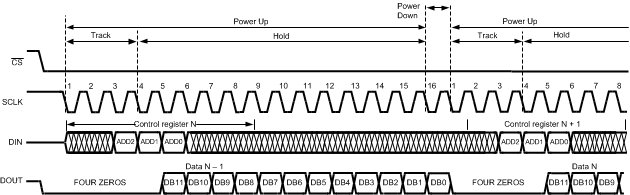Figure 1. ADC128S102 Operational Timing Diagram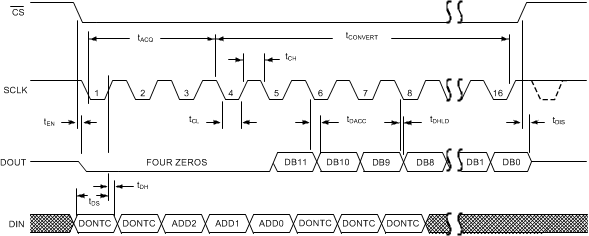Figure 2. ADC128S102 Serial Timing DiagramFigure 3. SCLK and CS Timing Parameters

### 6.9 Typical Characteristics

TA = 25°C, fSAMPLE = 1 MSPS, fSCLK = 16 MHz, fIN = 40.2 kHz unless otherwise stated.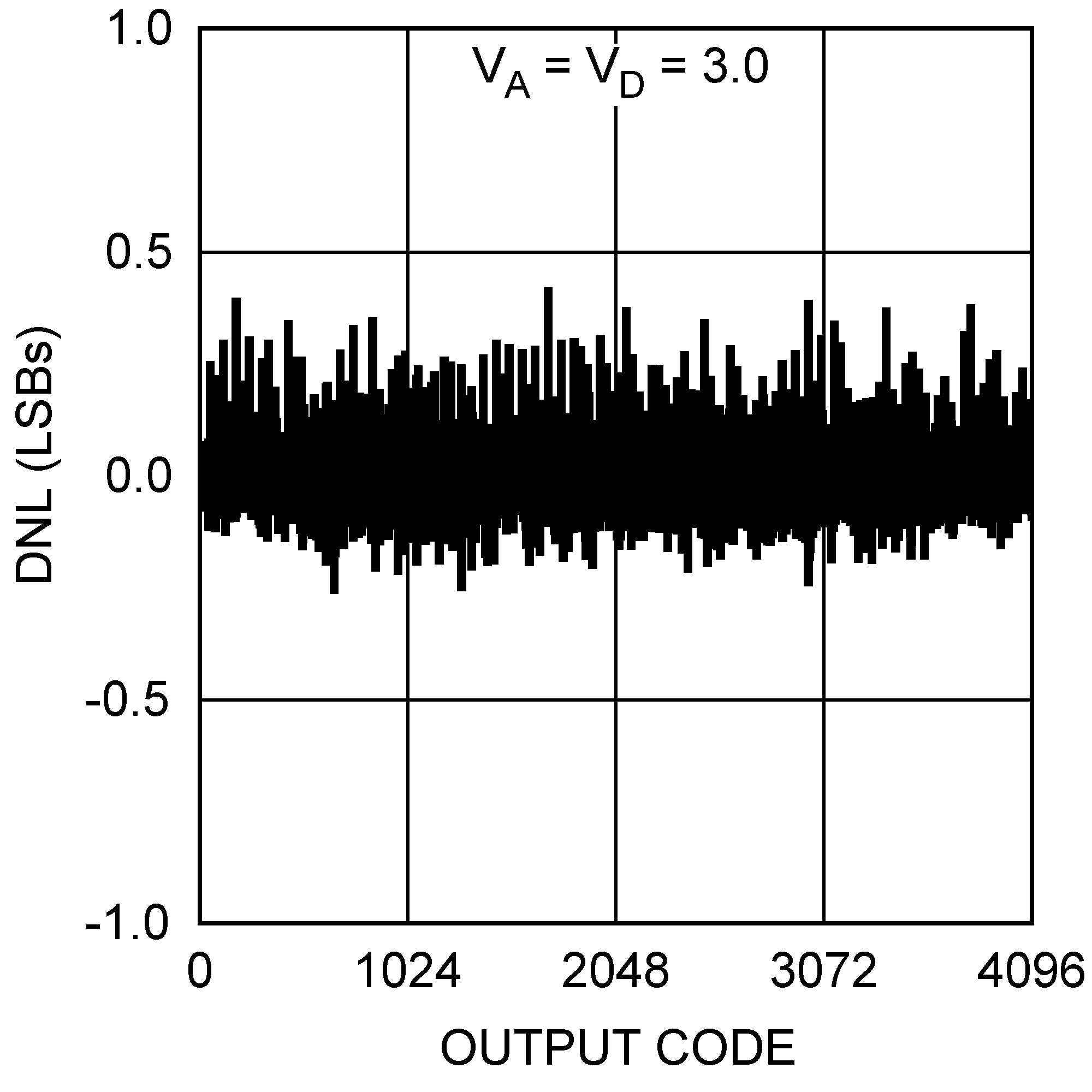Figure 4. DNL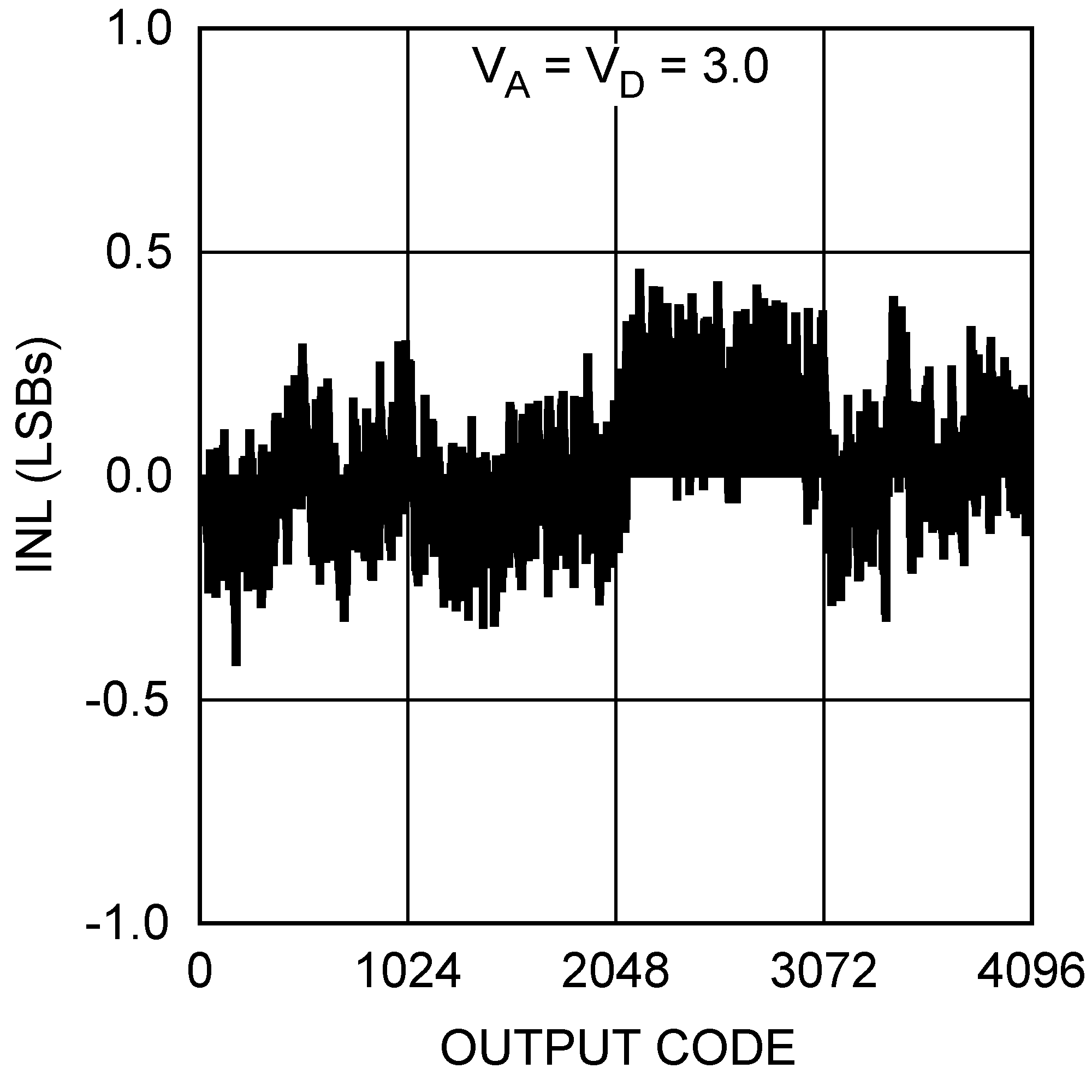Figure 6. INL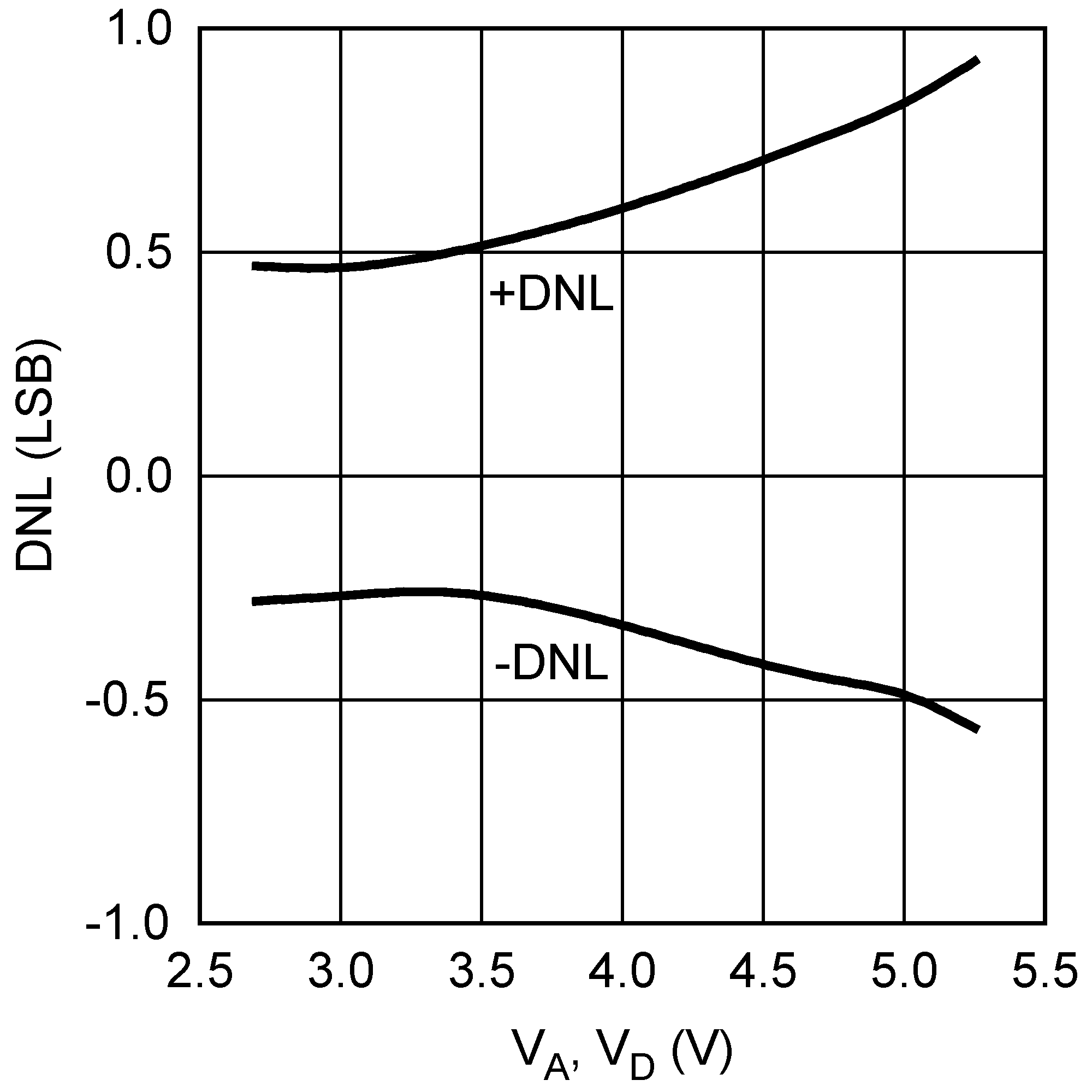Figure 8. DNL vs Supply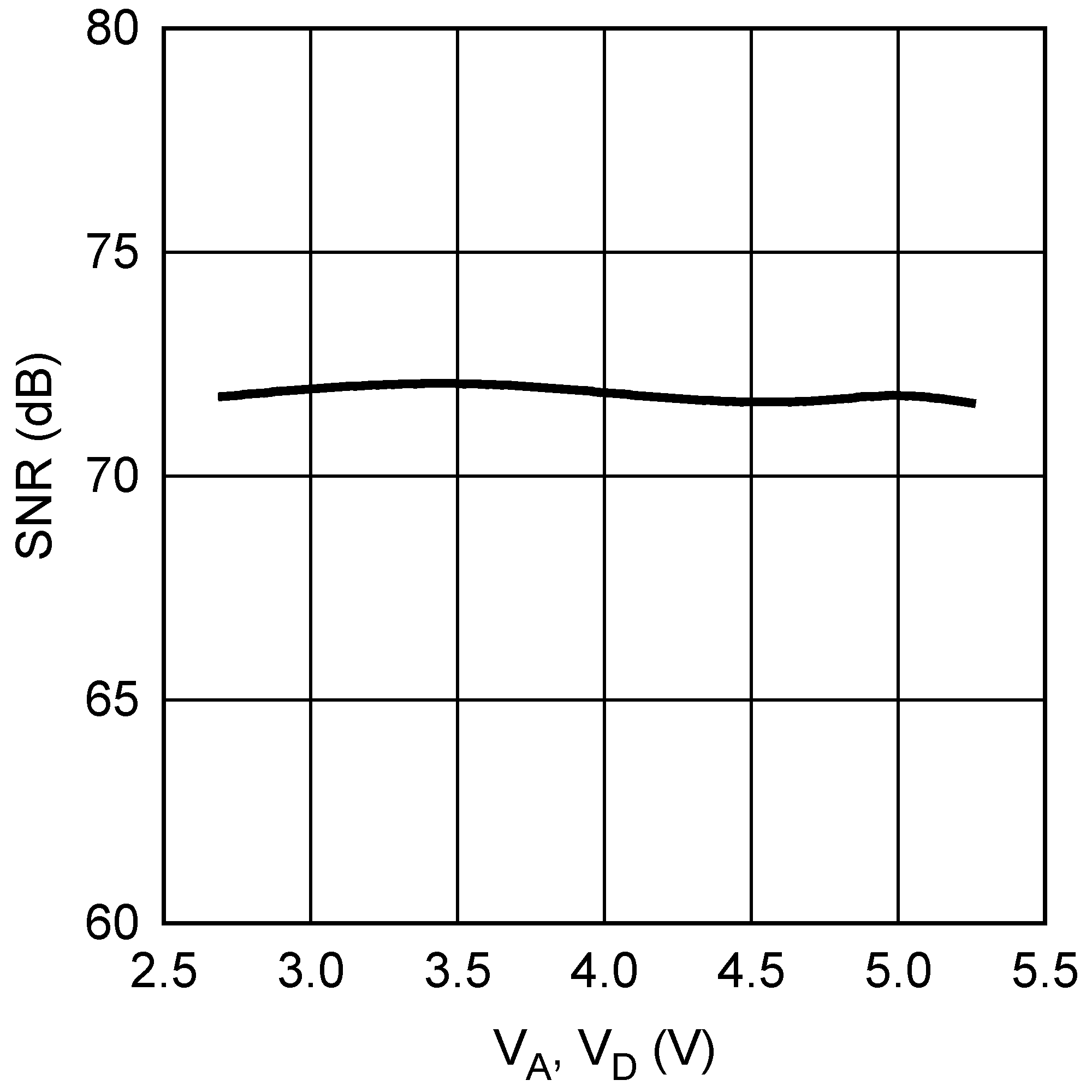Figure 10. SNR vs Supply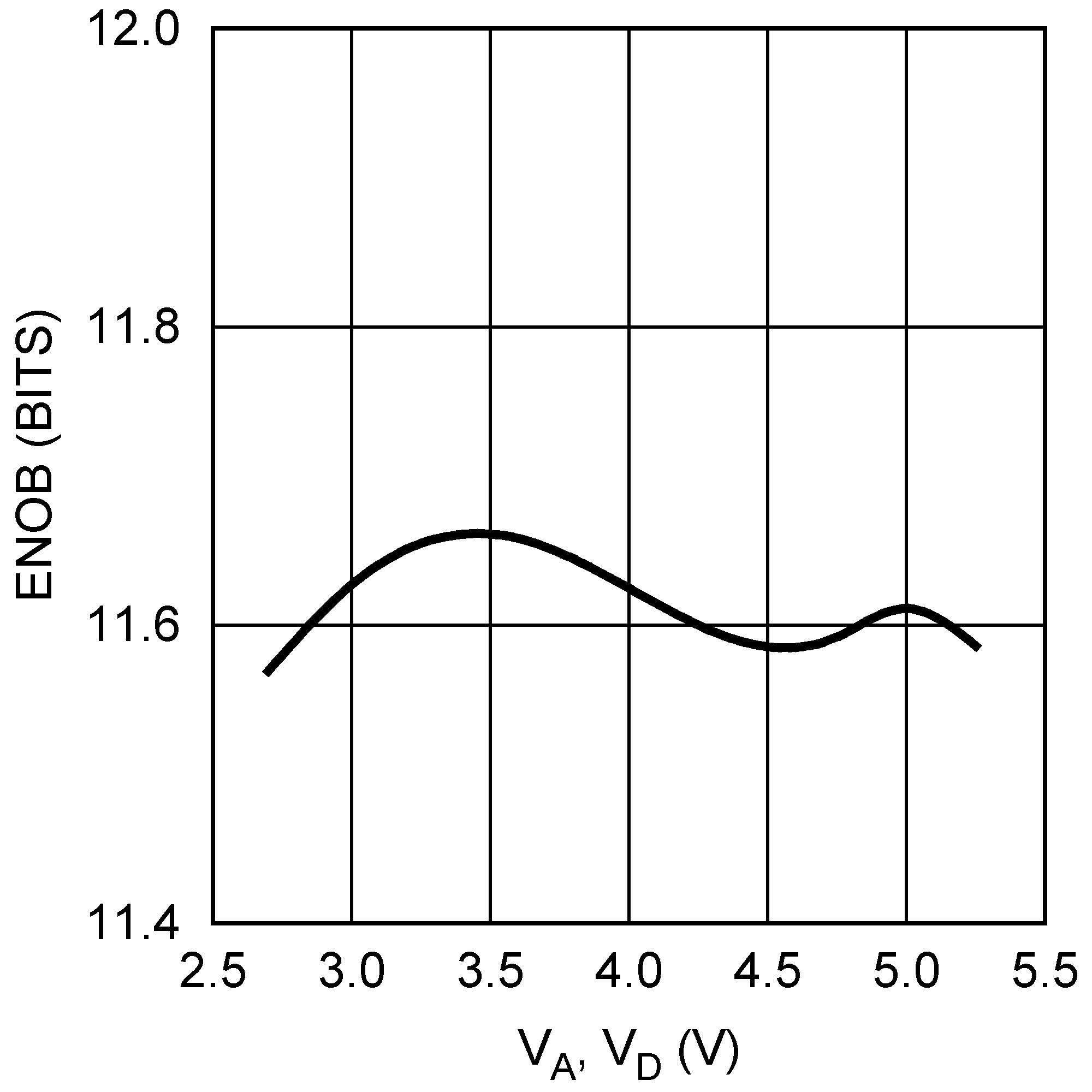Figure 12. ENOB vs Supply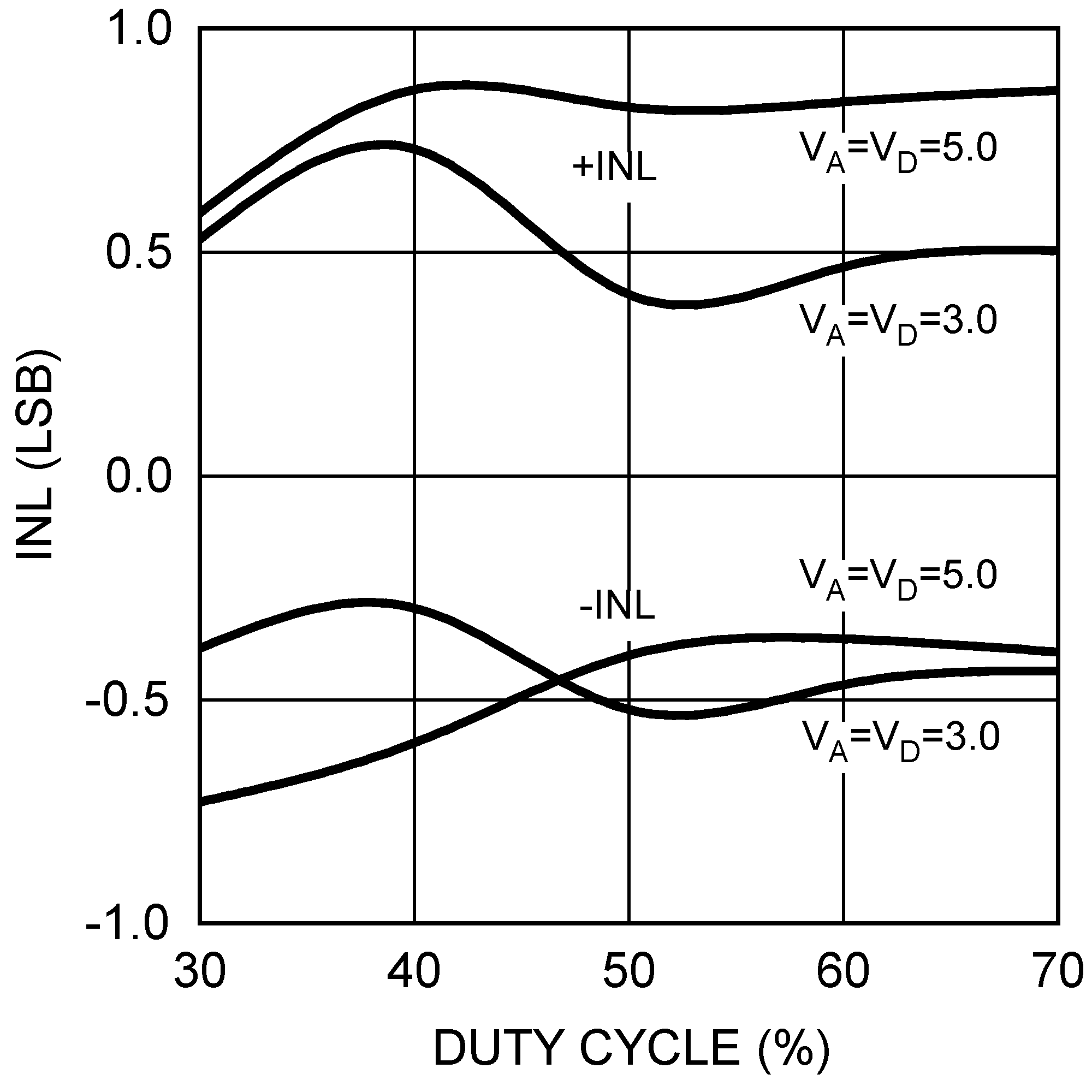Figure 14. INL vs SCLK Duty Cycle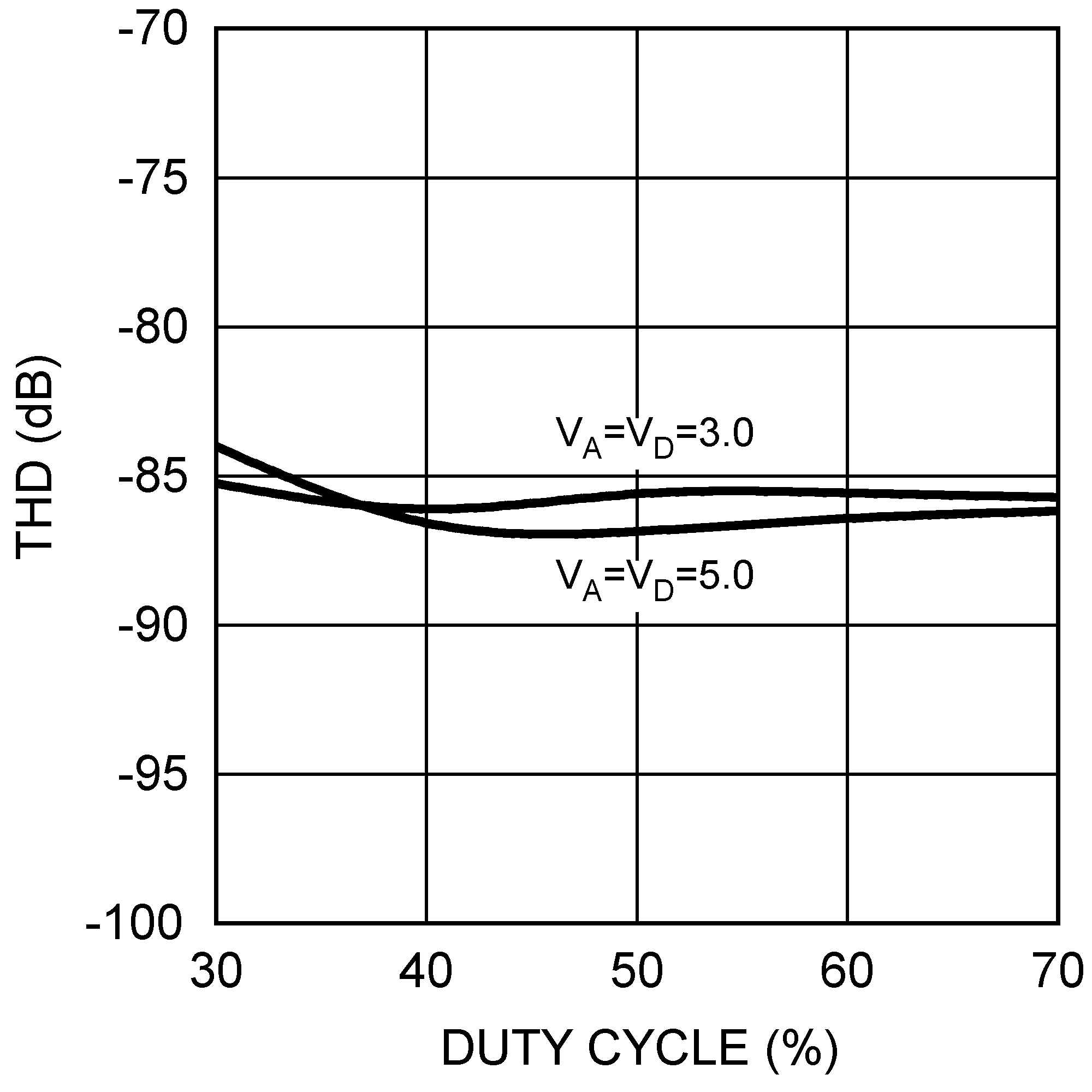Figure 16. THD vs SCLK Duty Cycle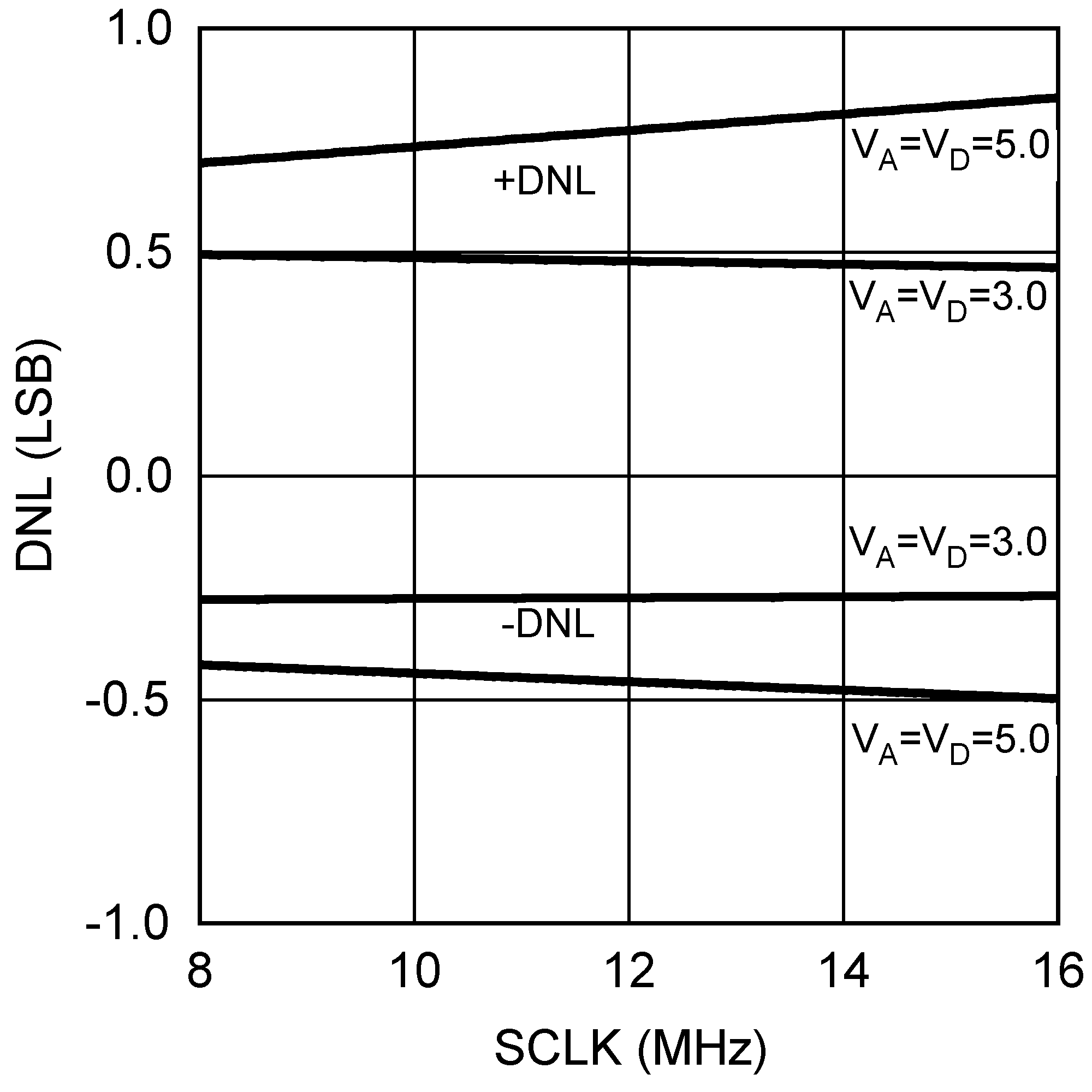Figure 18. DNL vs SCLK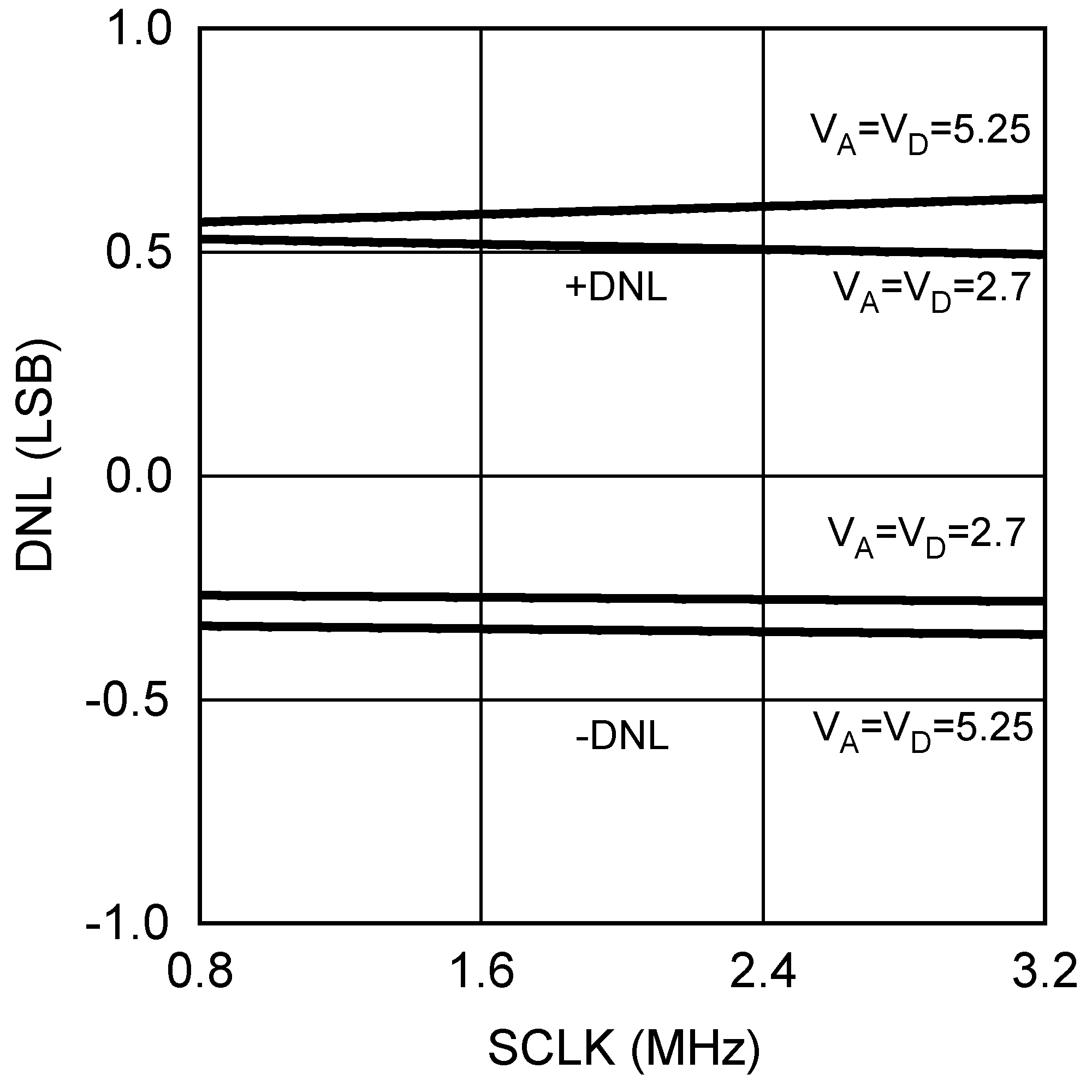Figure 20. DNL vs SCLK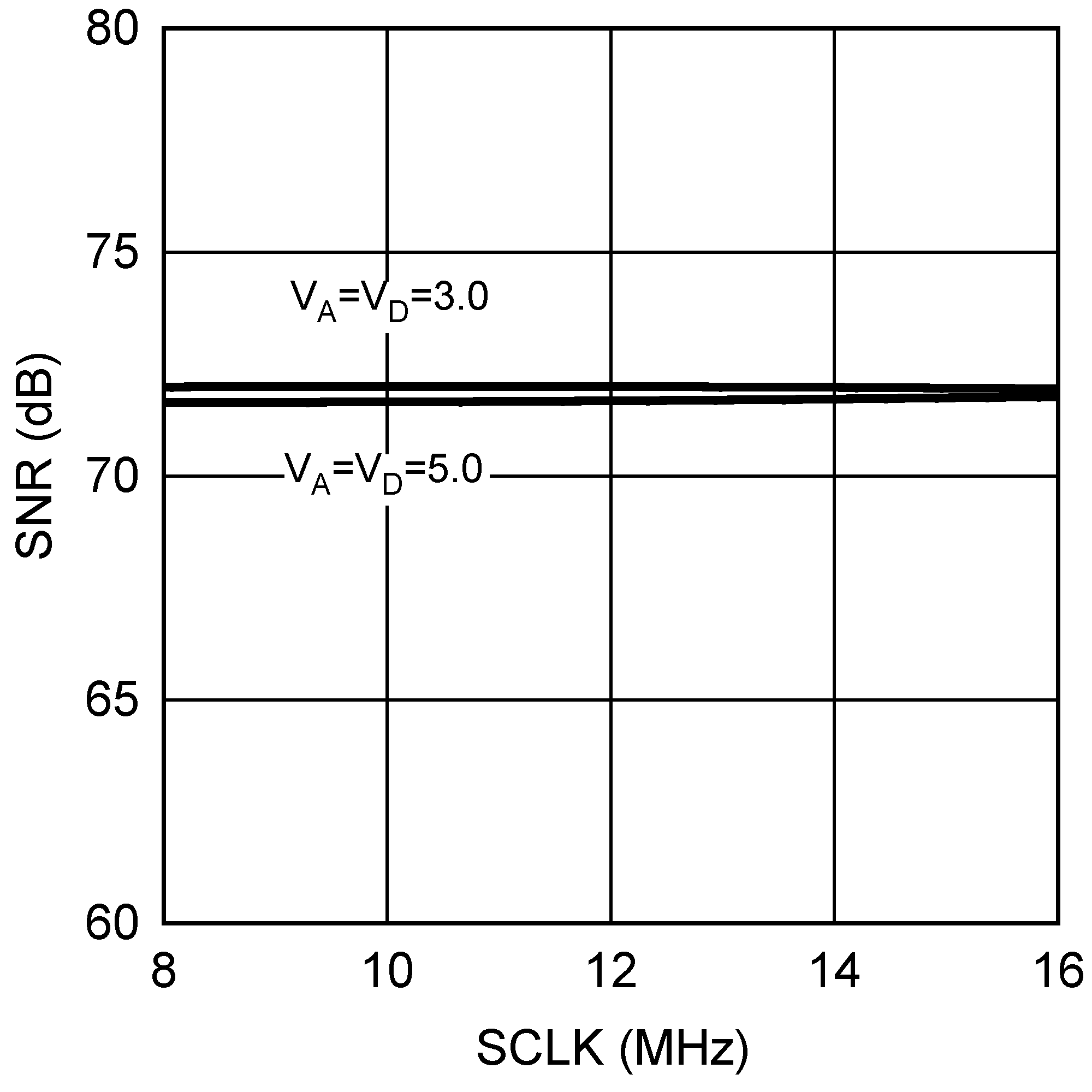Figure 22. SNR vs SCLK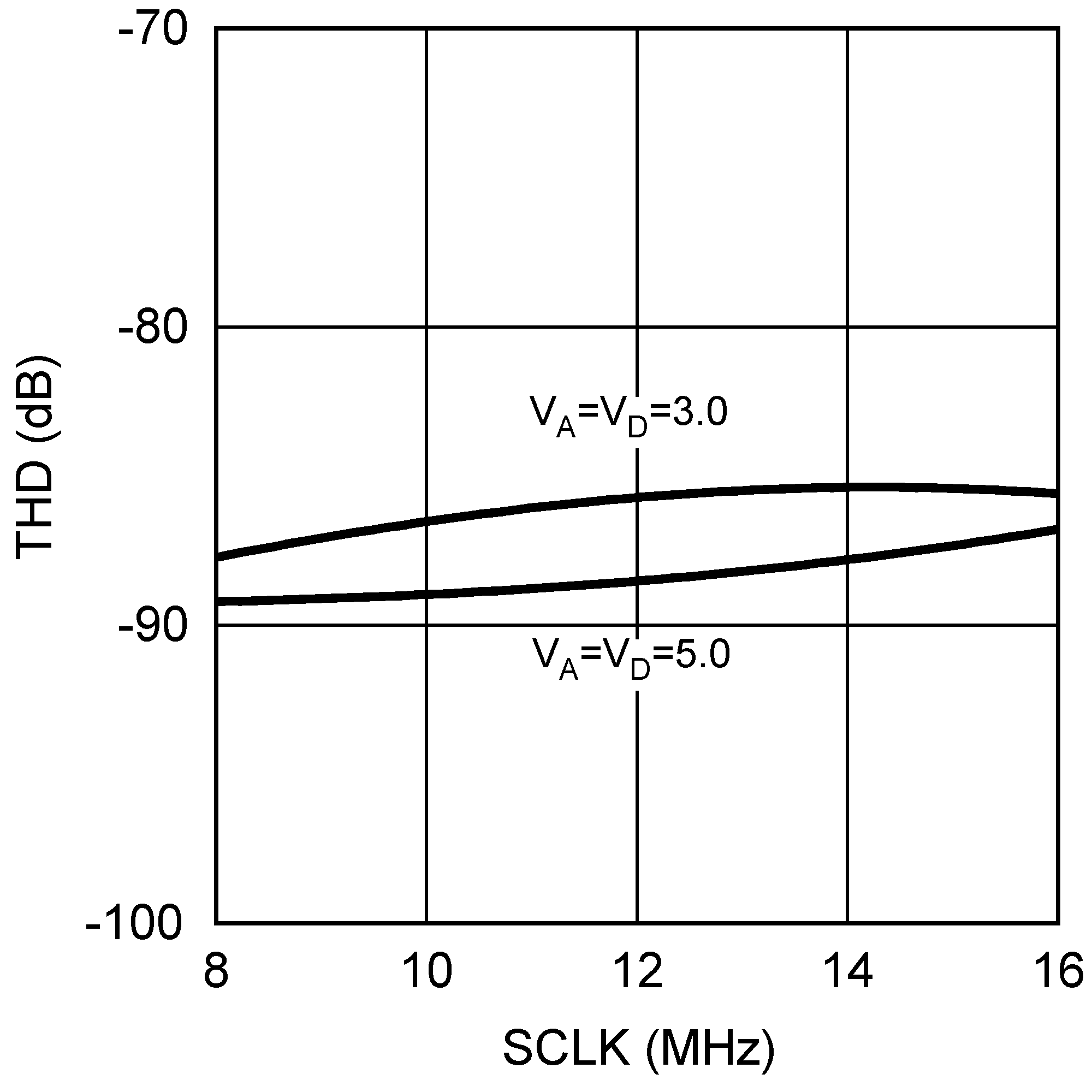Figure 24. THD vs SCLK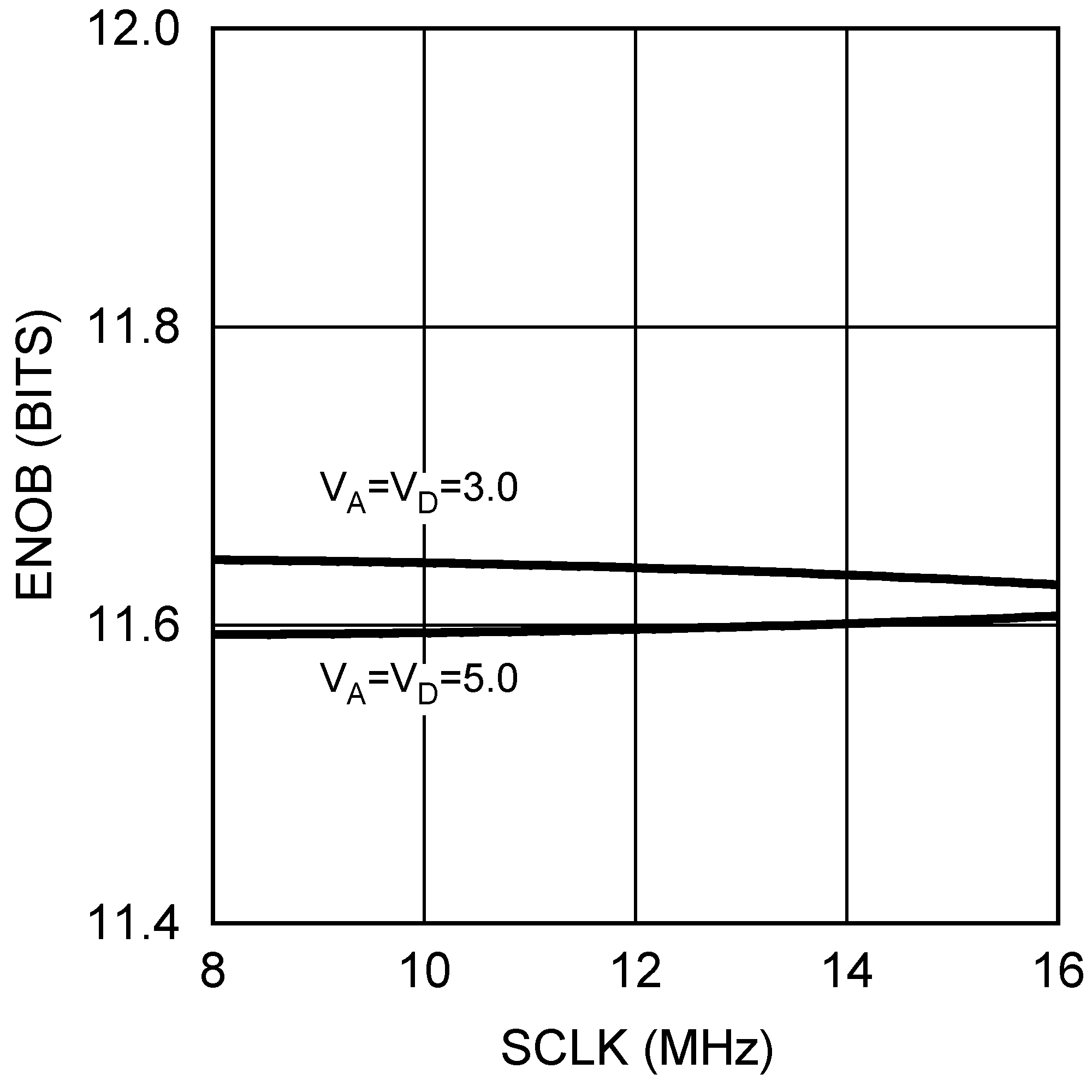Figure 26. ENOB vs SCLK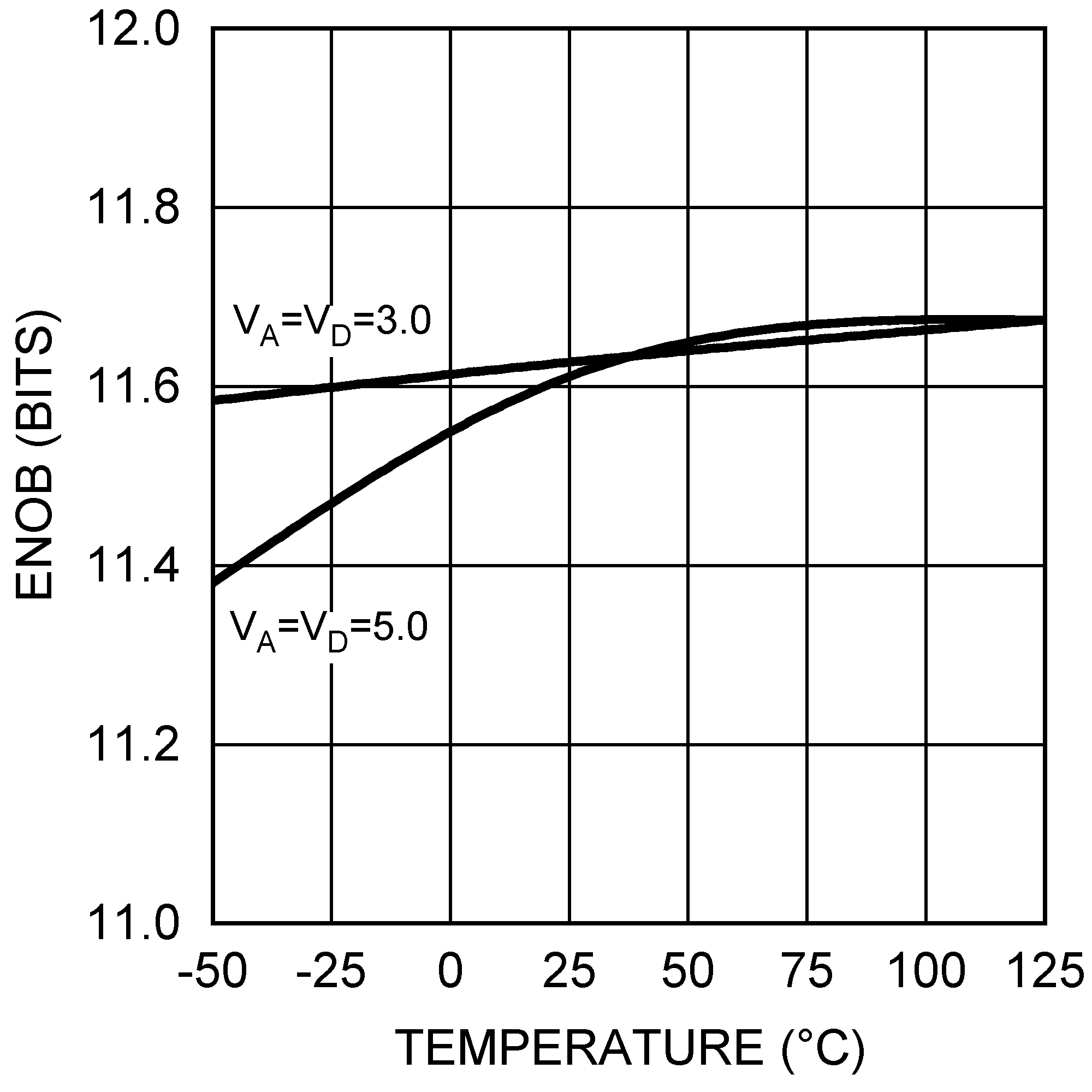Figure 28. ENOB vs Temperature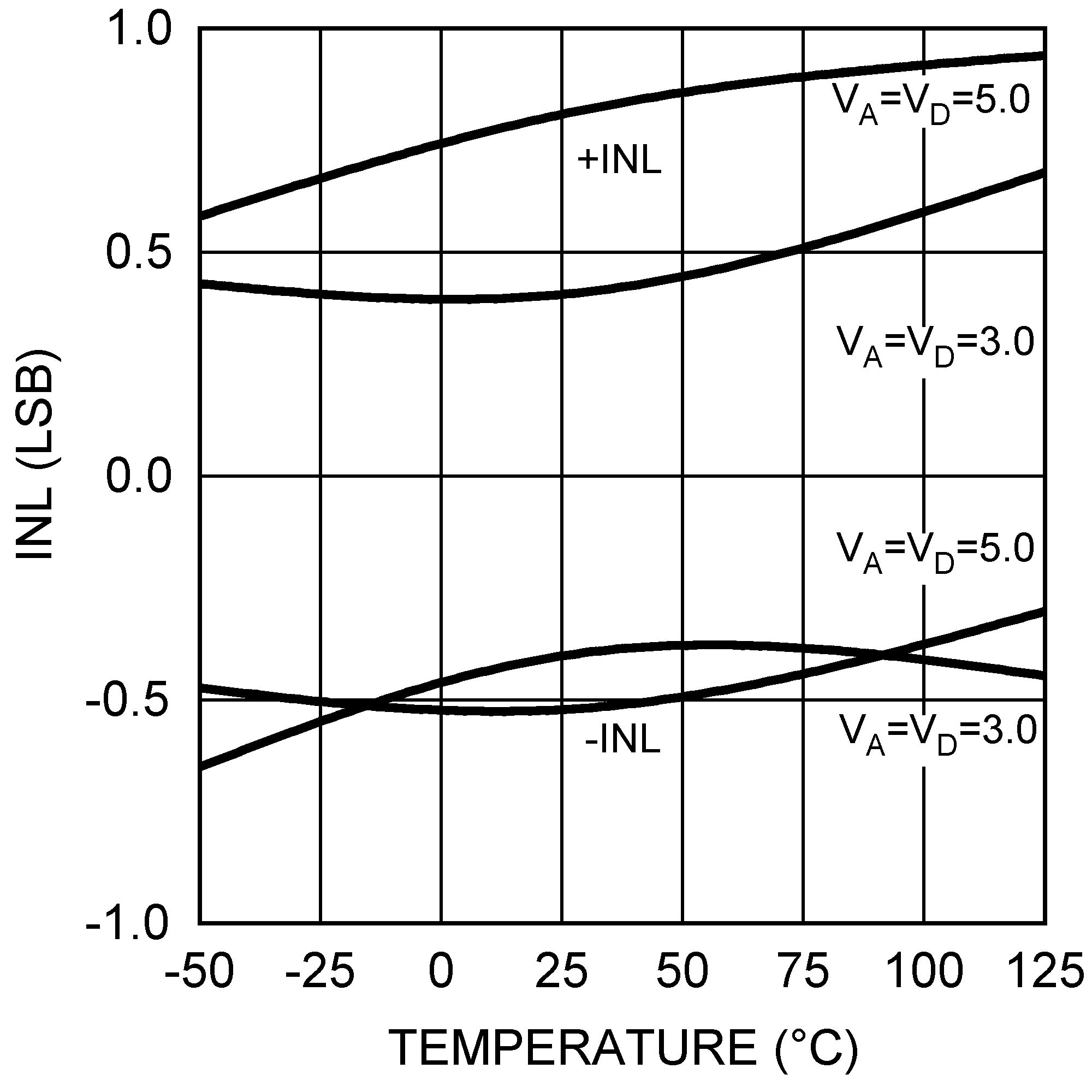Figure 30. INL vs Temperature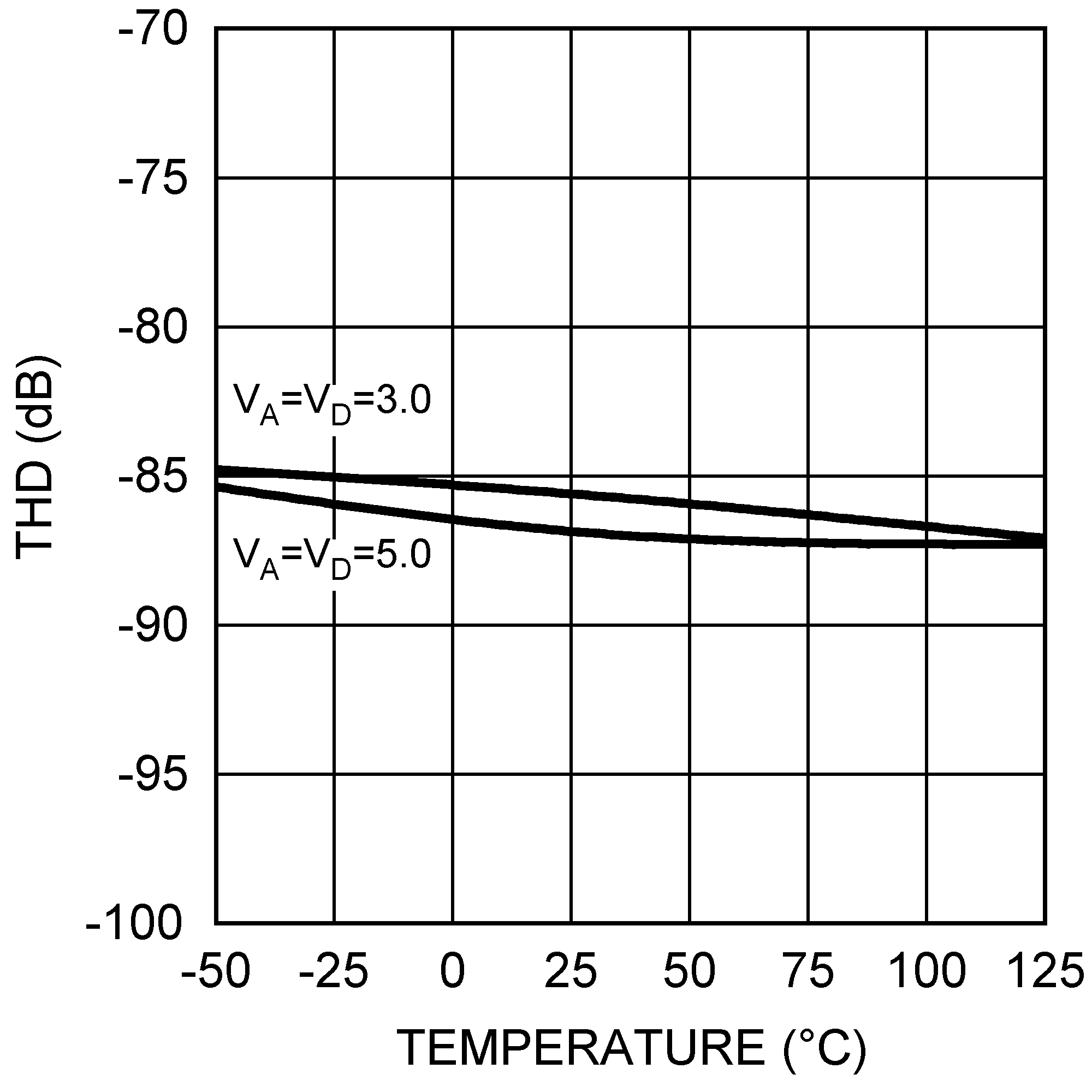Figure 32. THD vs Temperature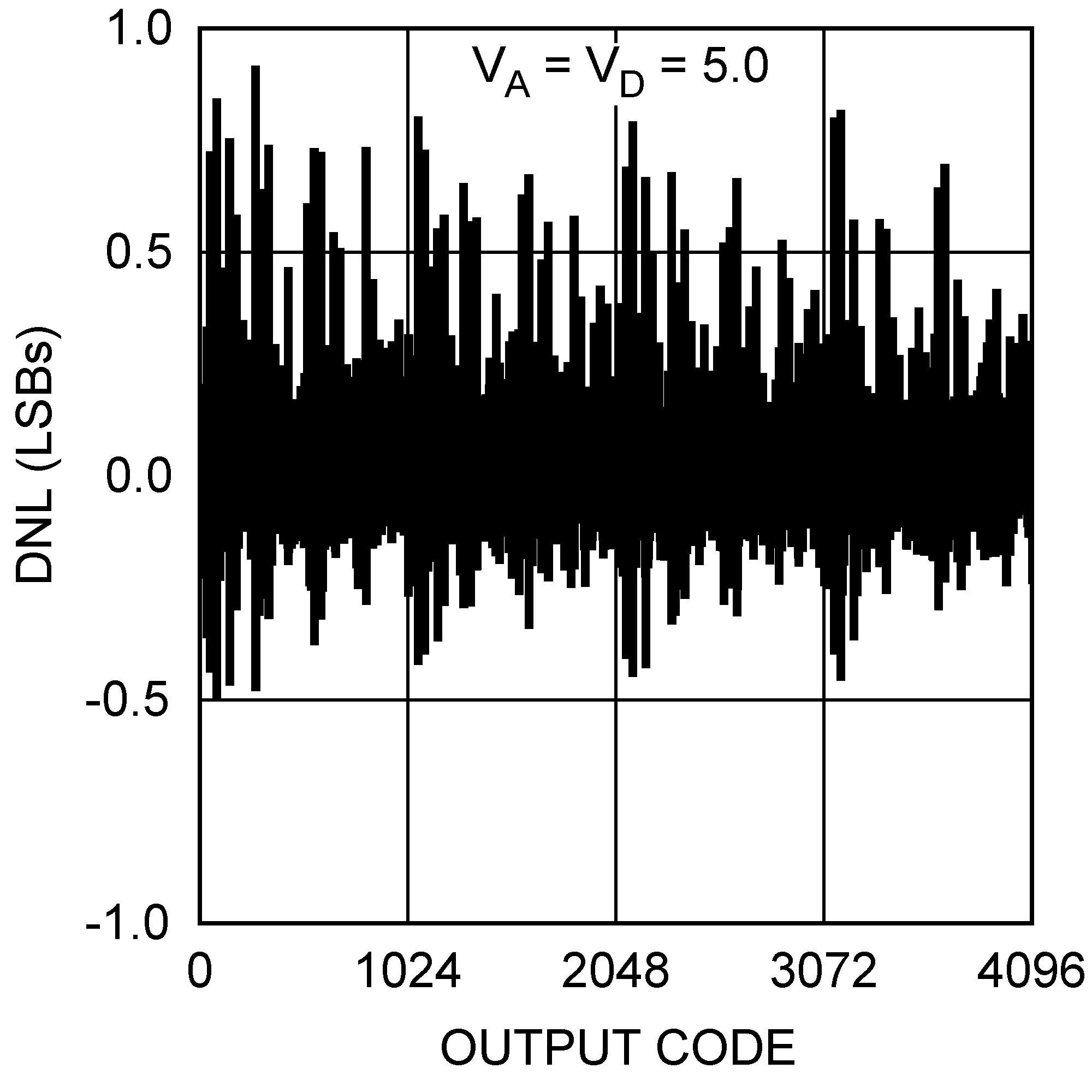Figure 5. DNL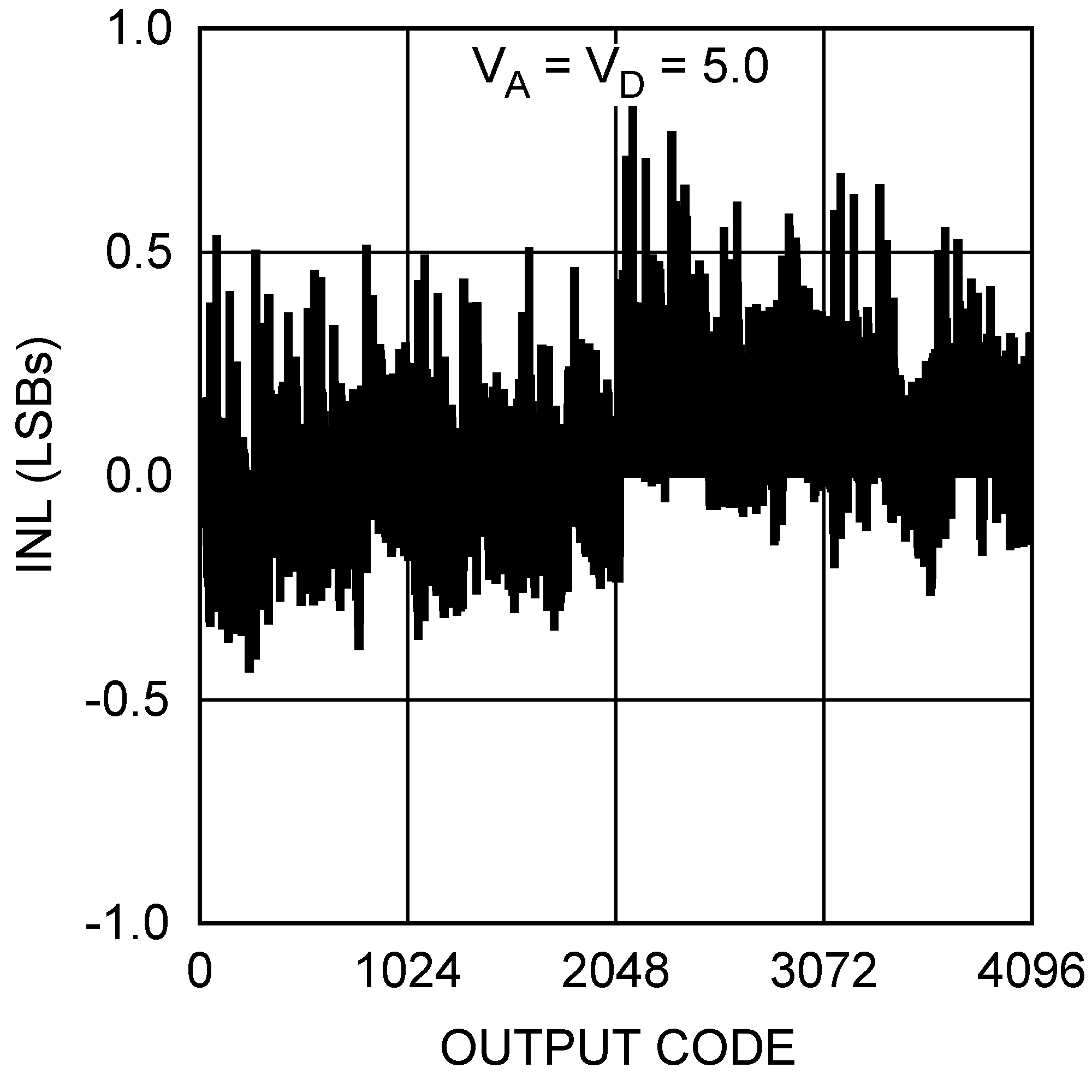Figure 7. INL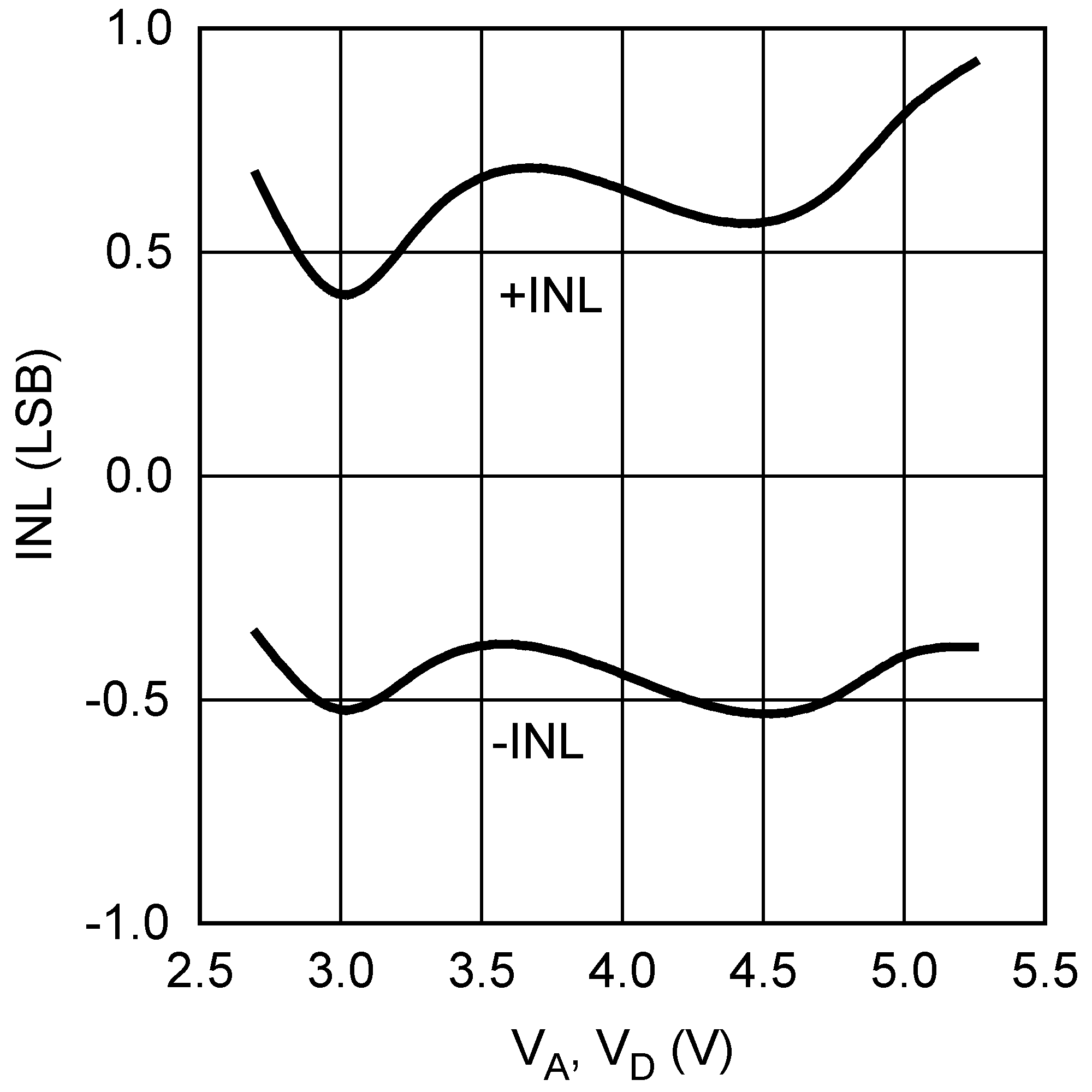Figure 9. INL vs Supply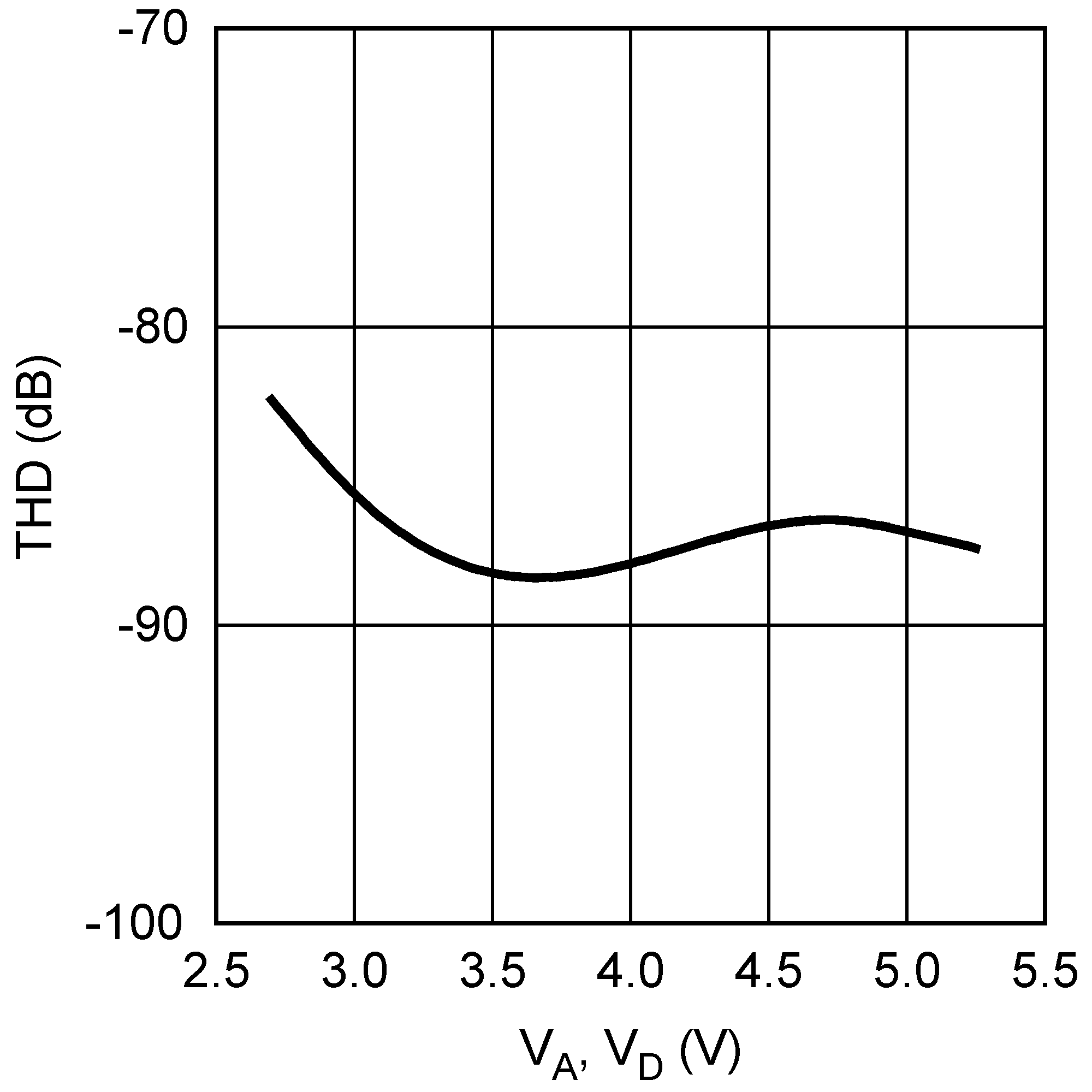Figure 11. THD vs Supply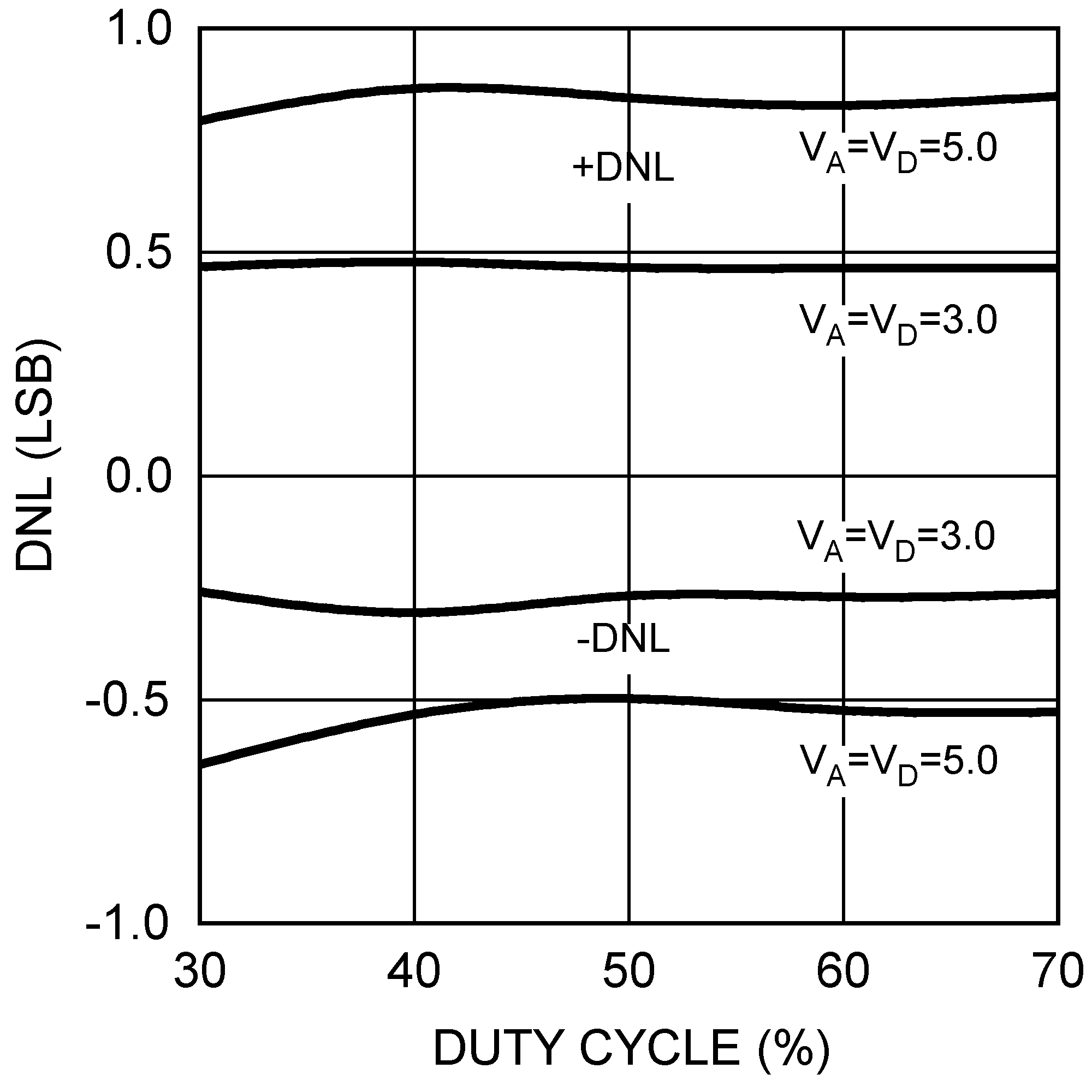Figure 13. DNL vs SCLK Duty Cycle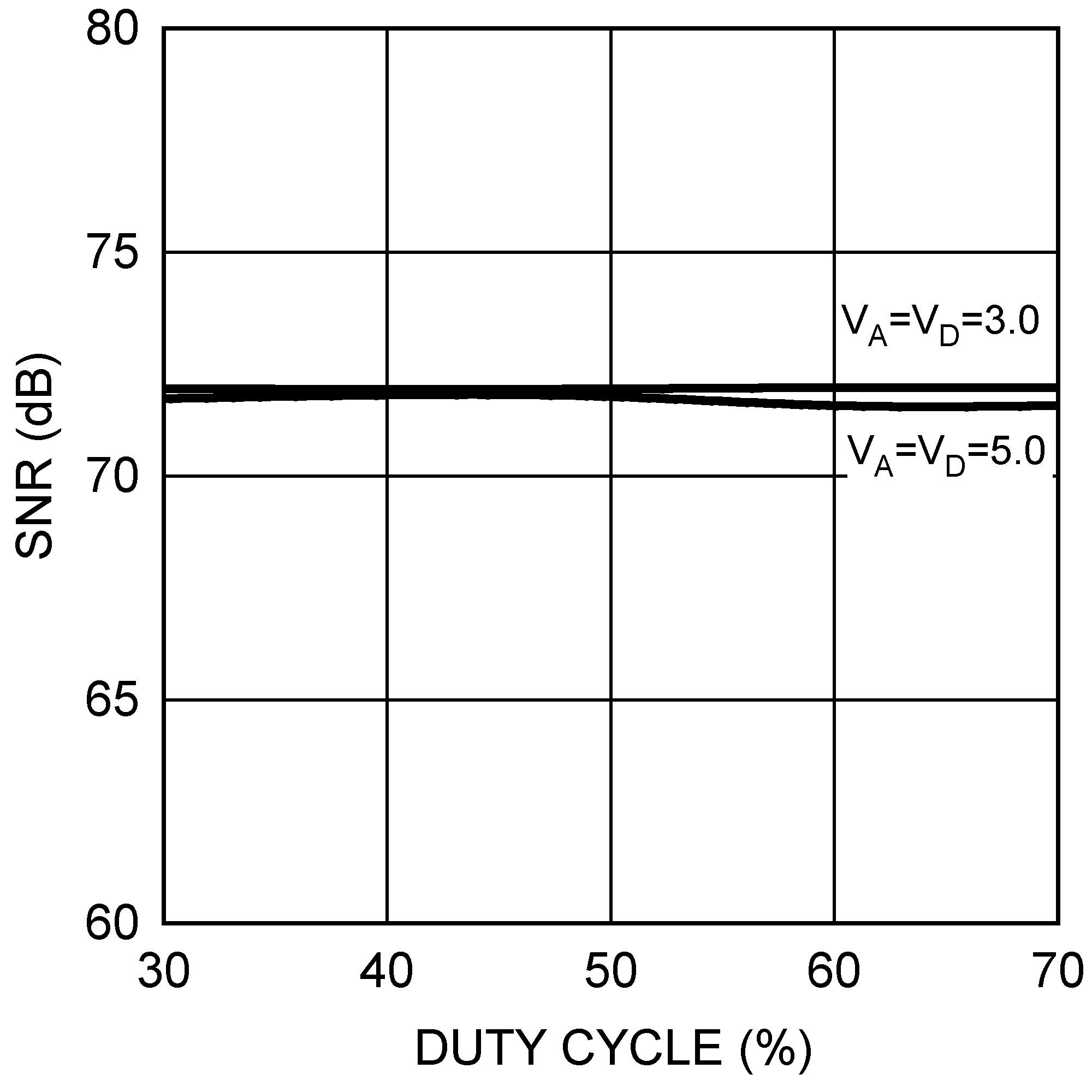Figure 15. SNR vs SCLK Duty Cycle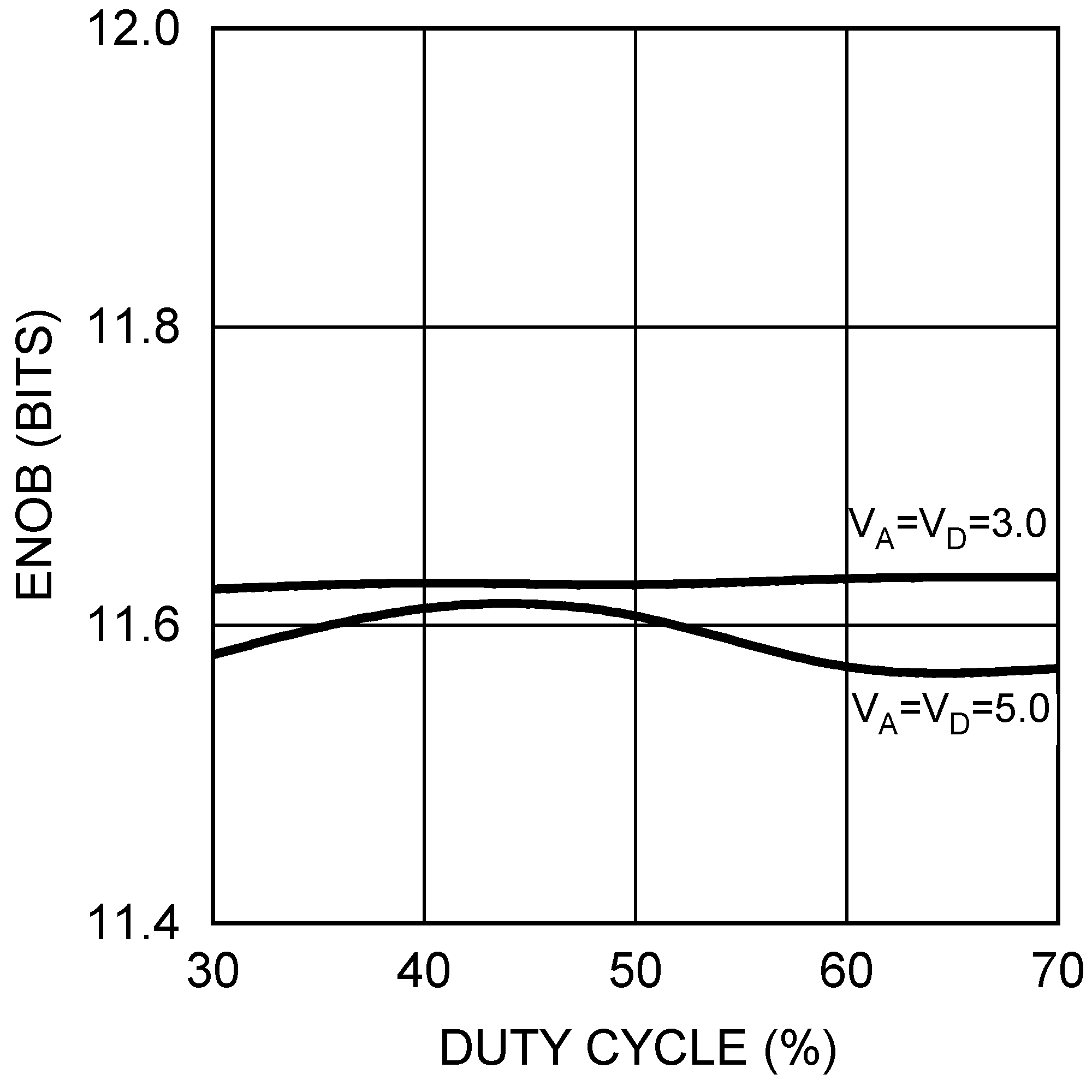Figure 17. ENOB vs SCLK Duty Cycle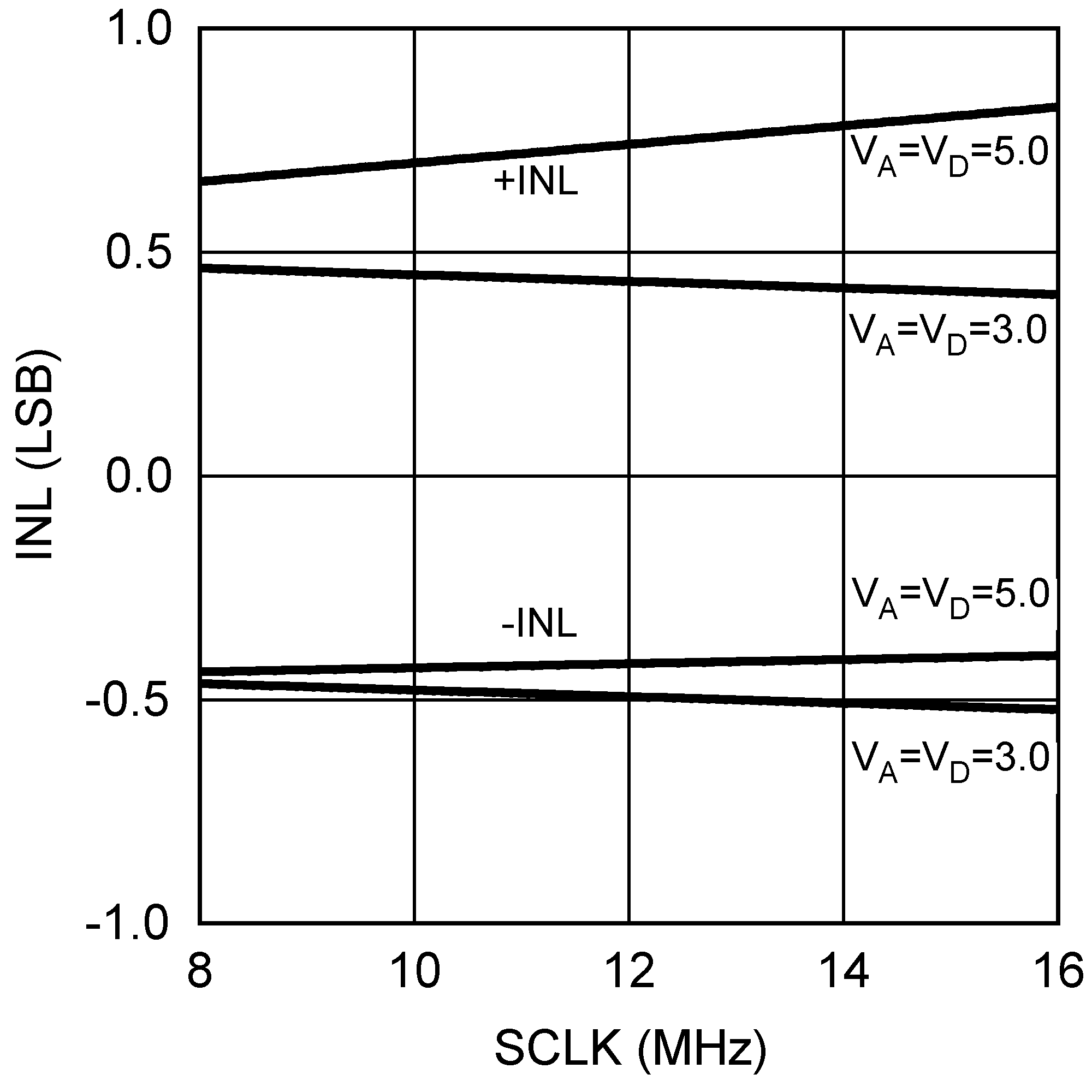Figure 19. INL vs SCLK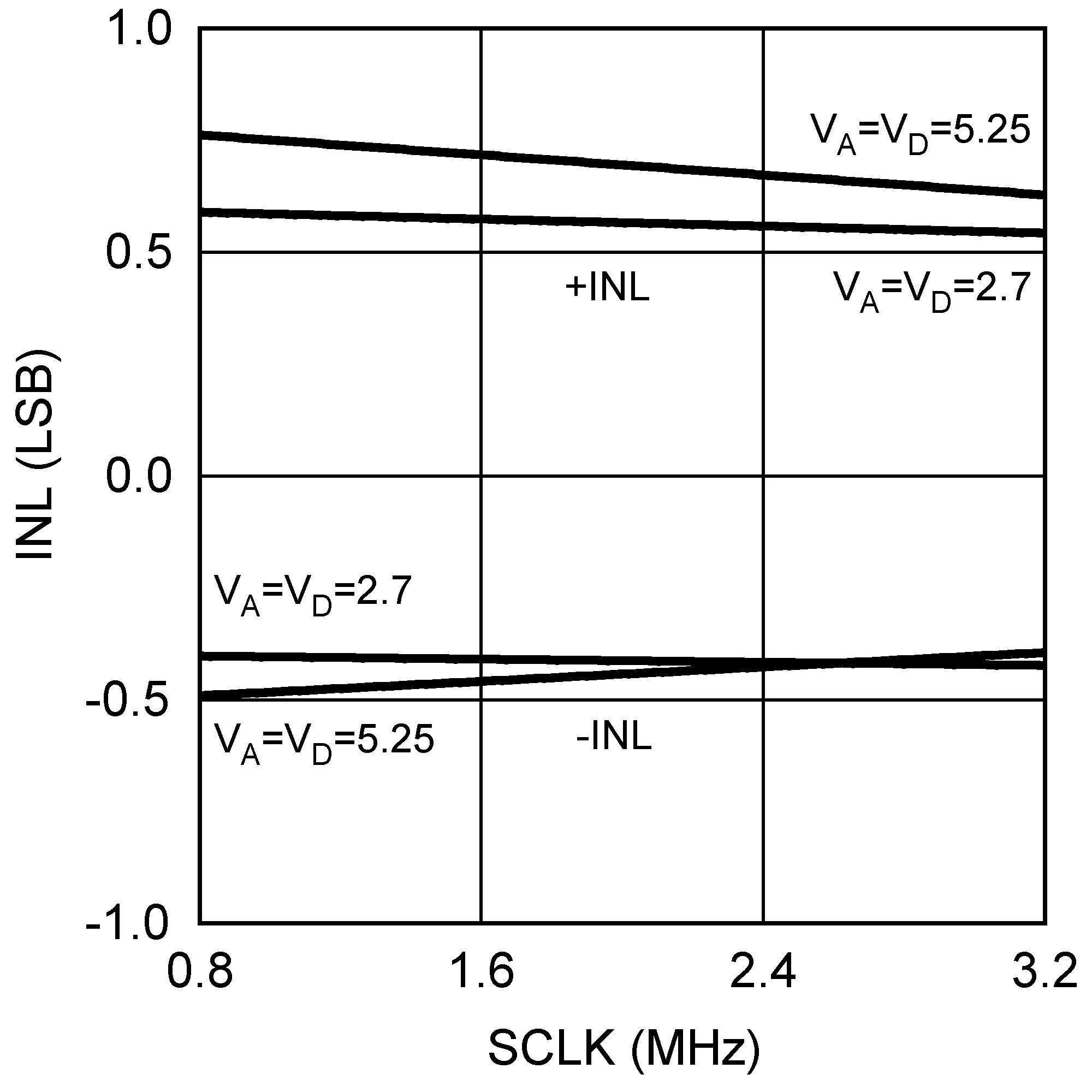Figure 21. INL vs SCLK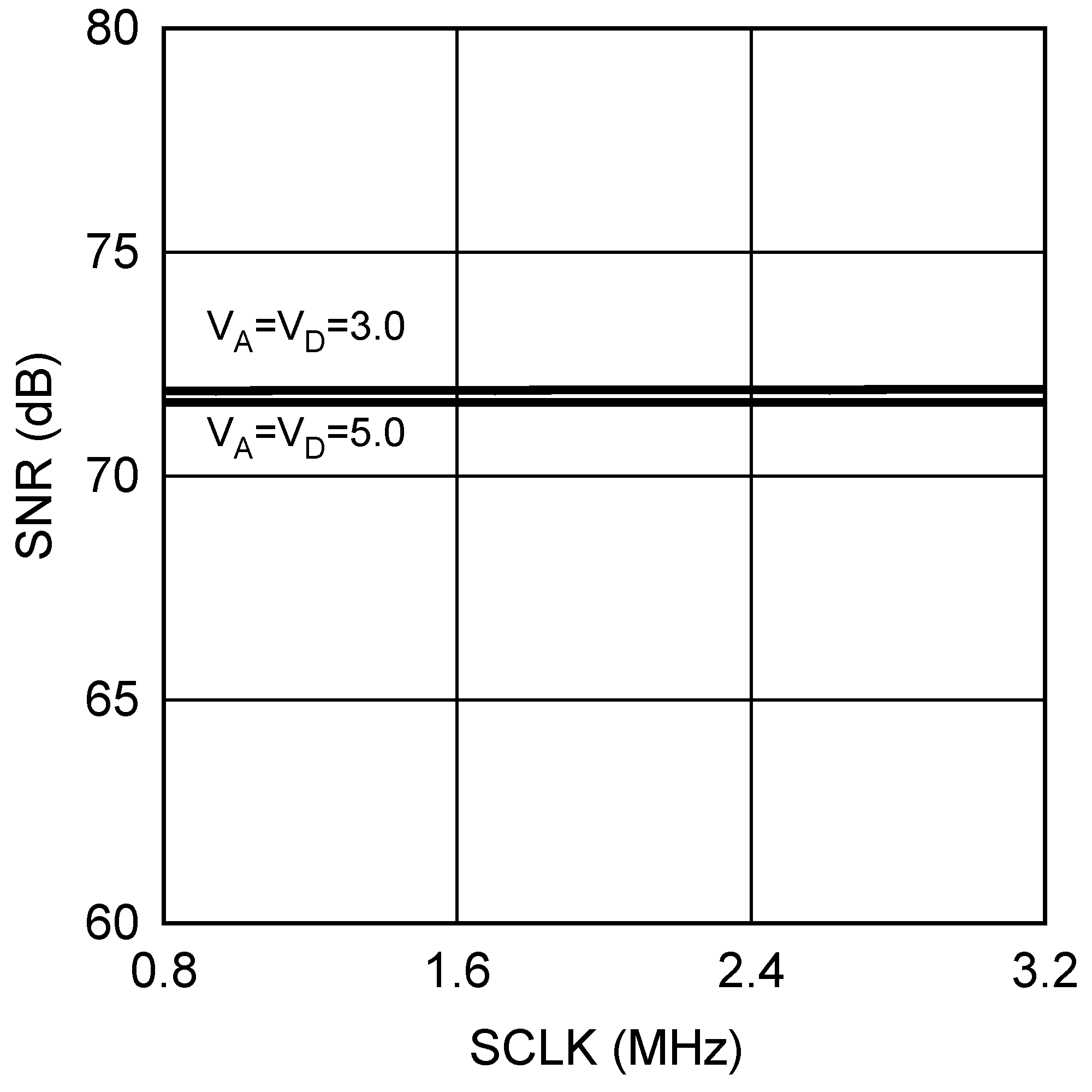Figure 23. SNR vs SCLK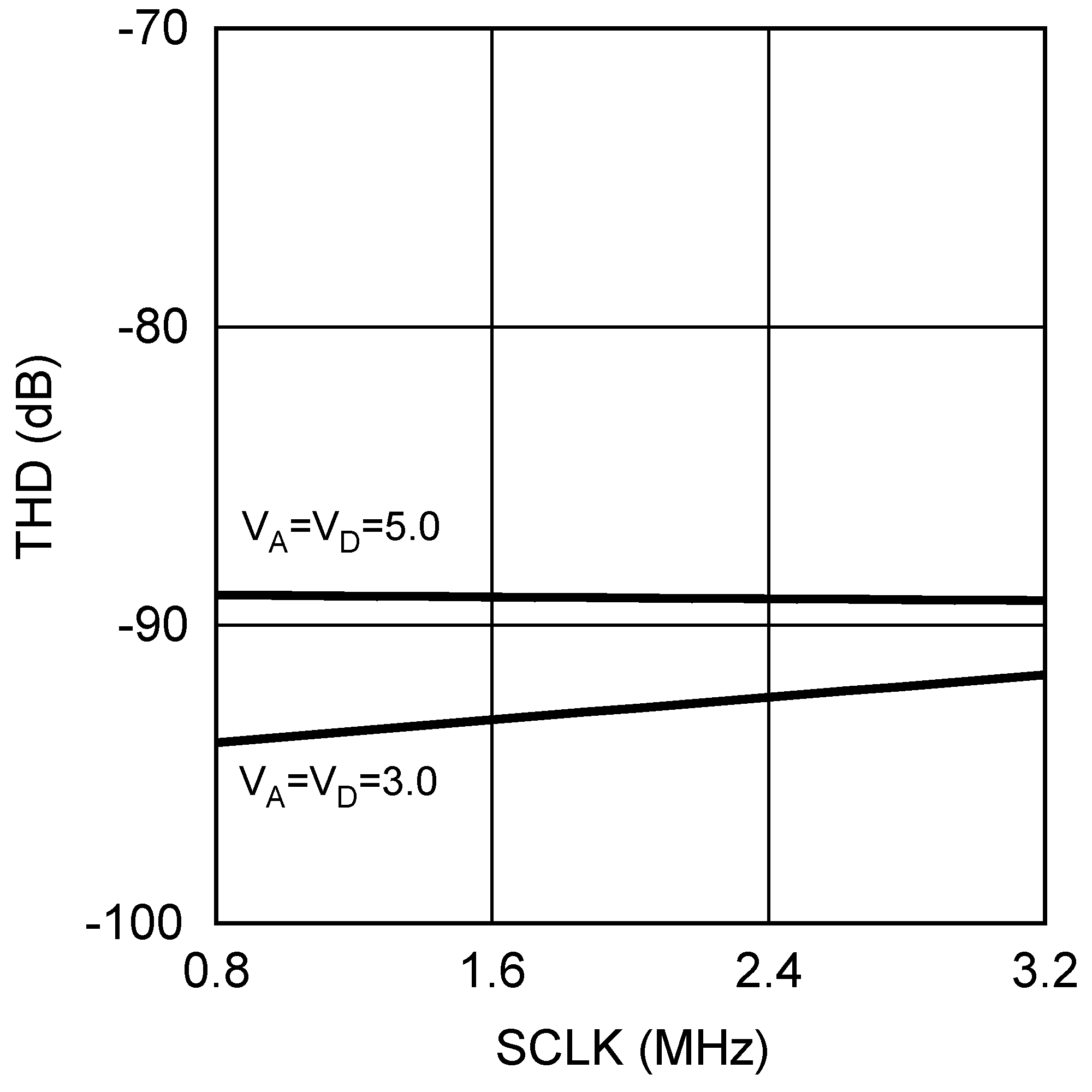Figure 25. THD vs SCLK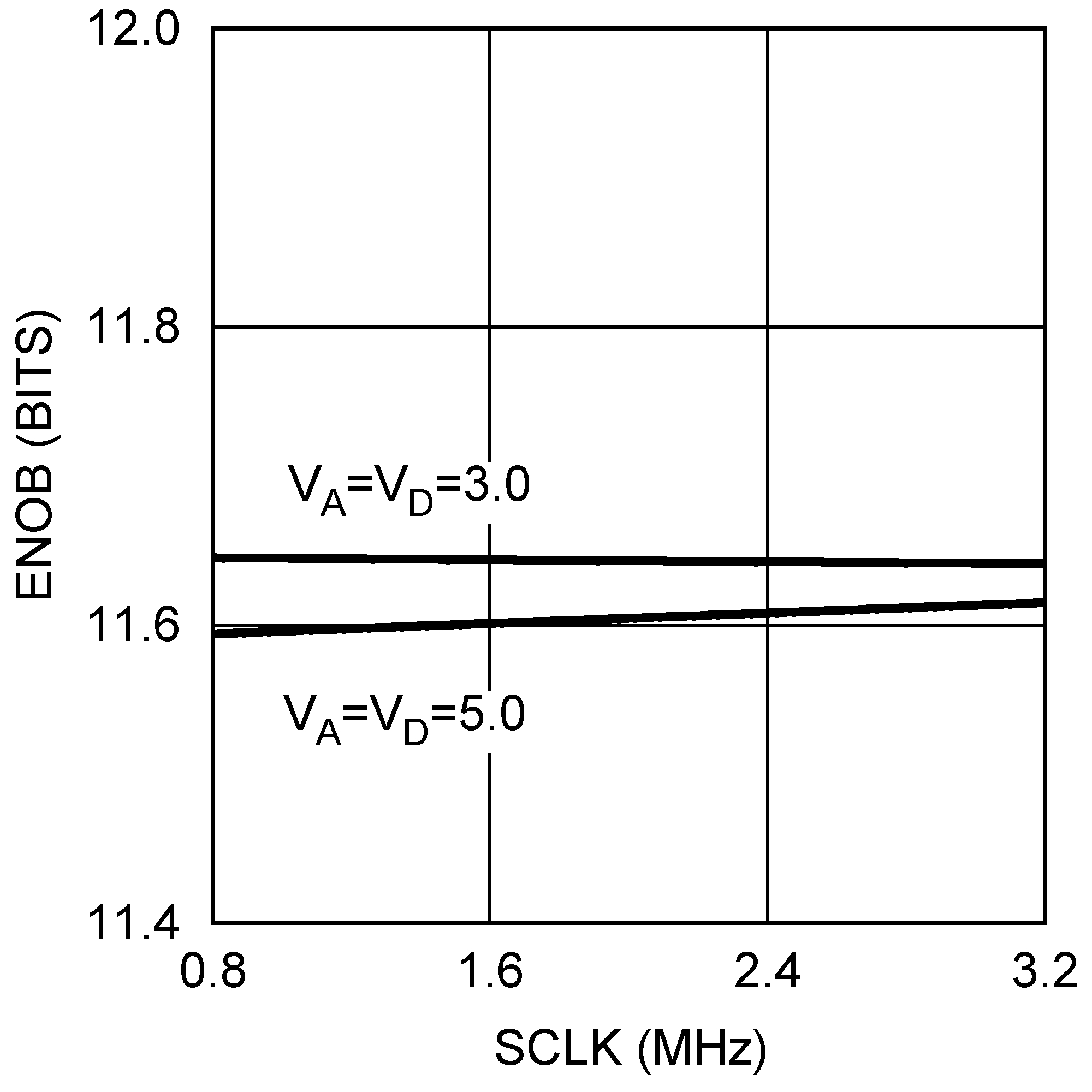Figure 27. ENOB vs SCLK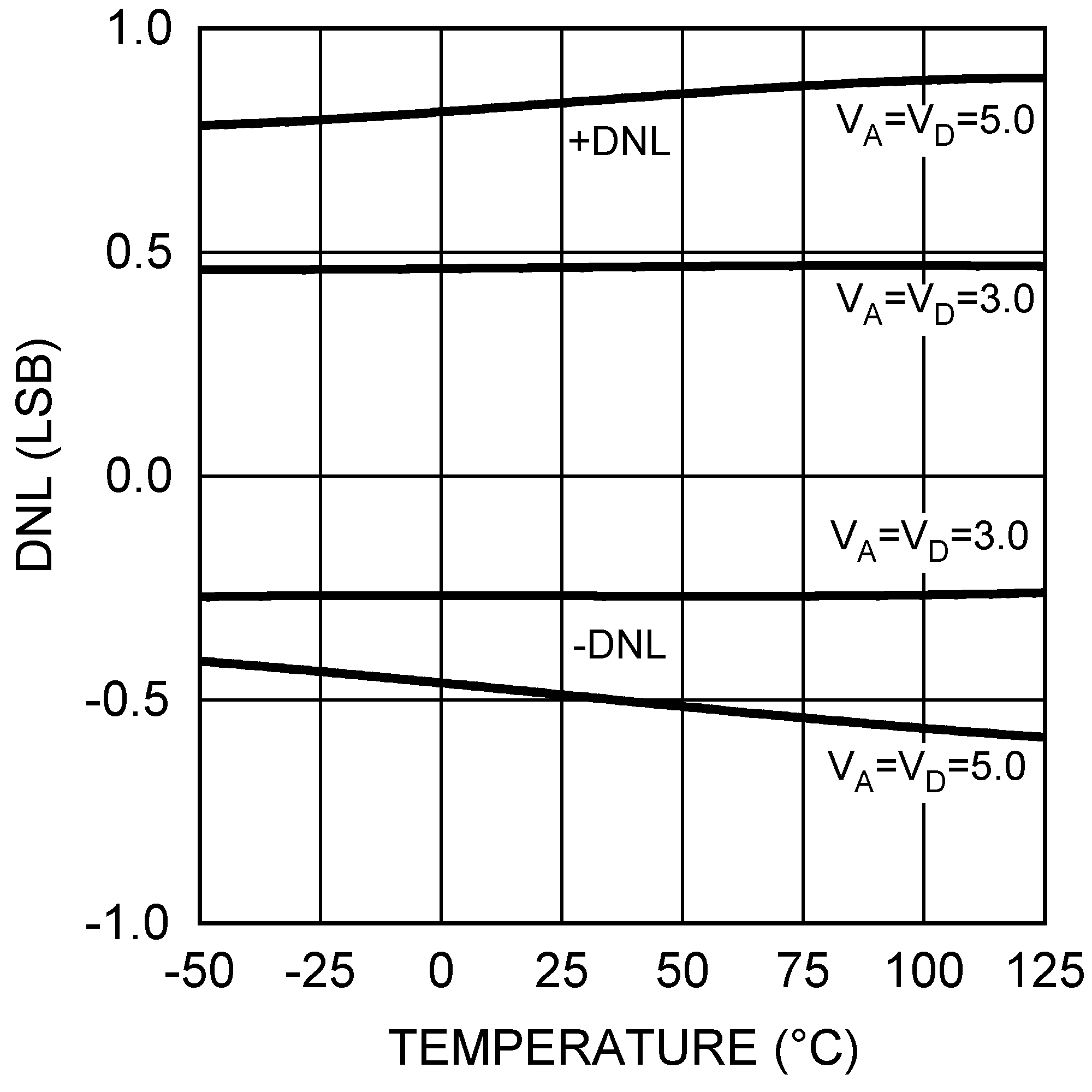Figure 29. DNL vs Temperature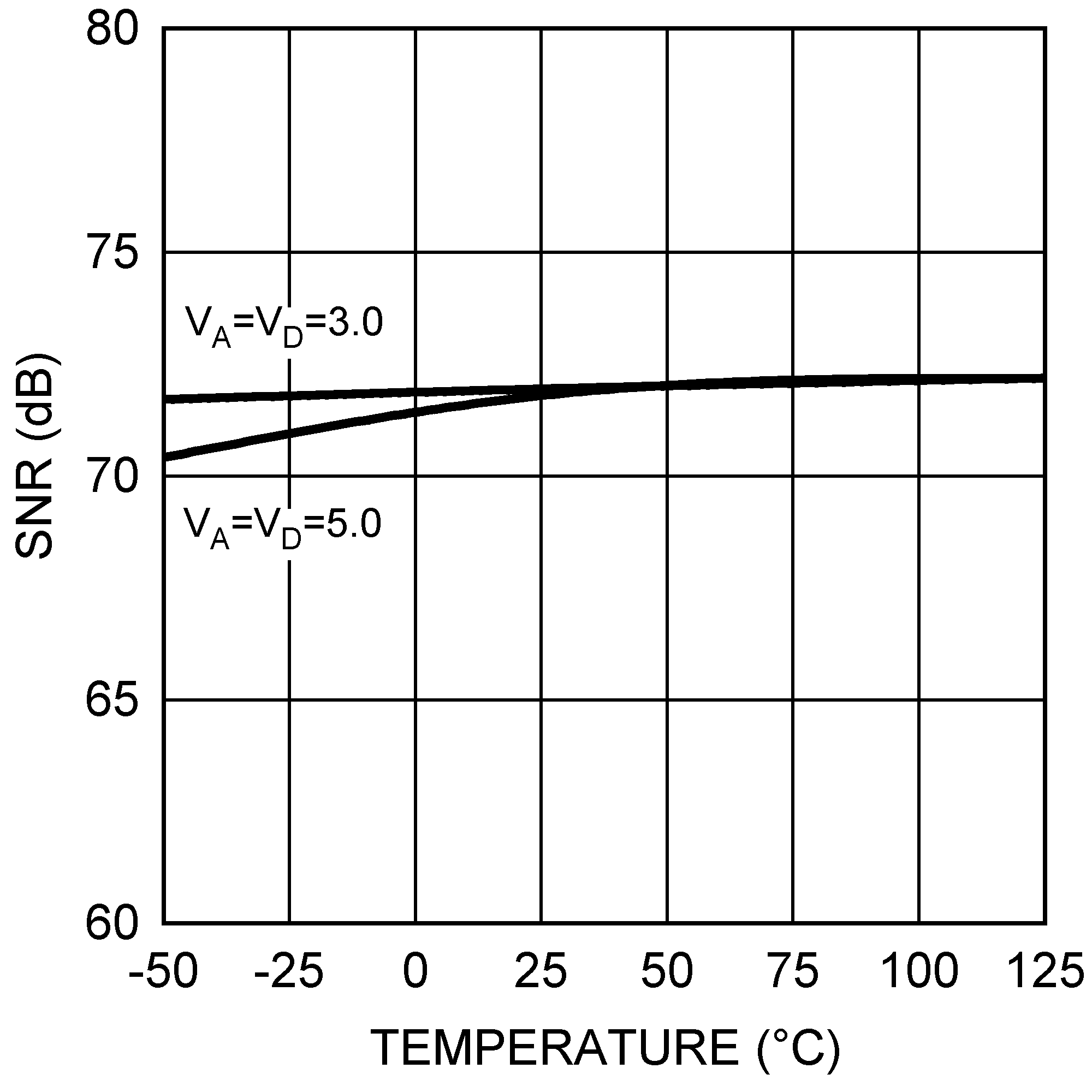Figure 31. SNR vs Temperature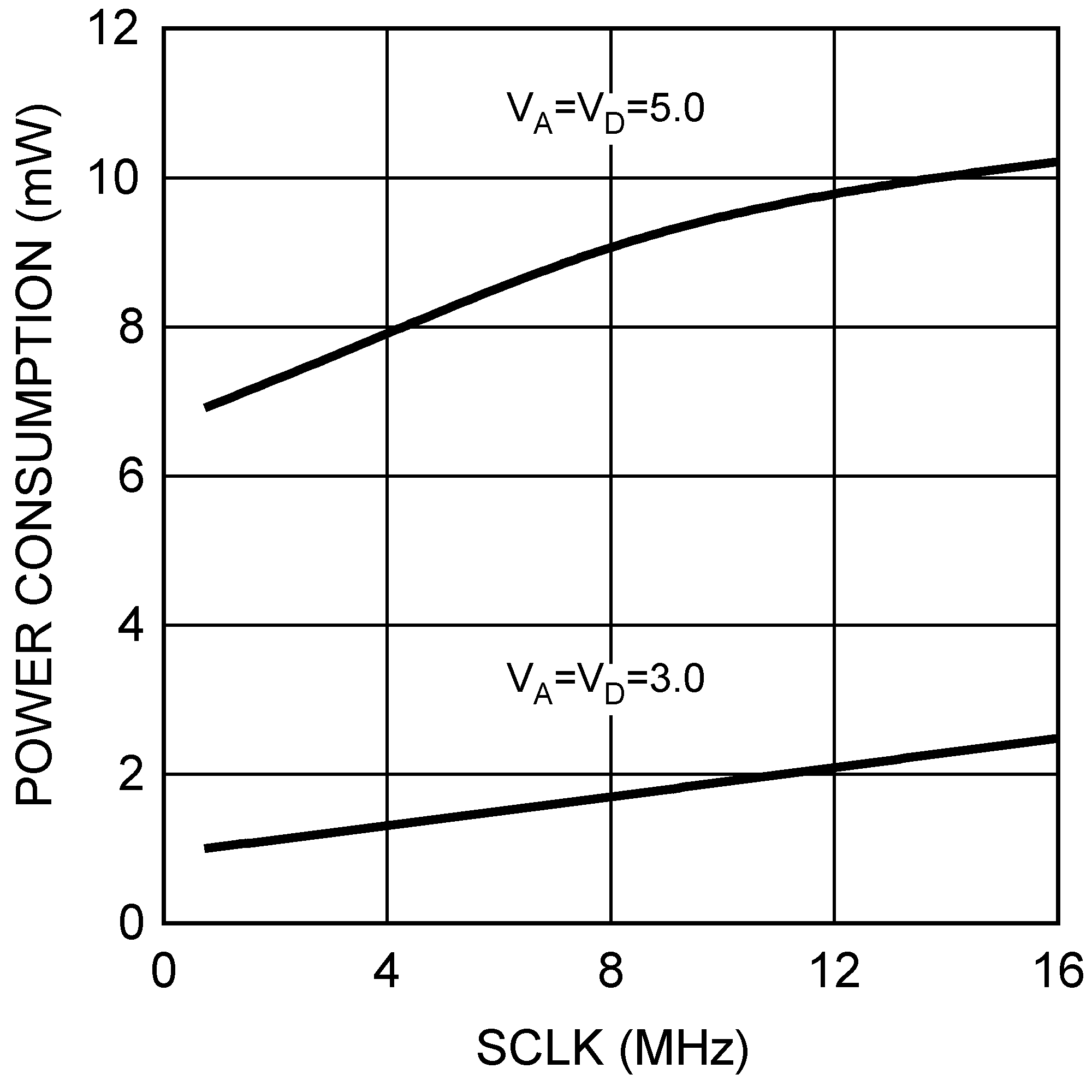Figure 33. Power Consumption vs SCLK